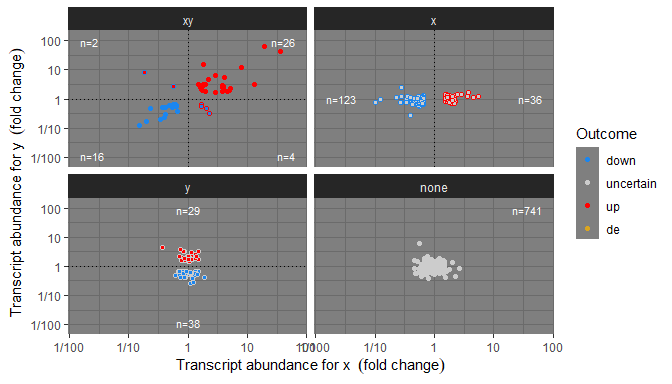# Model-Based Plot Annotations

## Preliminaries

We load all the packages used in the examples.

library(ggpmisc)
library(tibble)
library(dplyr)
library(quantreg)

eval_nlme <-  requireNamespace("nlme", quietly = TRUE)
if (eval_nlme) library(nlme)
eval_broom <-  requireNamespace("broom", quietly = TRUE)
if (eval_broom) library(broom)
eval_broom_mixed <-  requireNamespace("broom.mixed", quietly = TRUE)
if (eval_broom_mixed) library(broom.mixed)

As we will use text and labels on the plotting area we change the default theme to an uncluttered one.

old_theme <- theme_set(theme_bw())

# Introduction to computed annotations

Common plot annotations based on model fits are model equations, tests of significance and various indicators of goodness of fit. What annotations are most useful depend on whether both x and y are mapped to continuous variables, or y to a continuous variable, and x to a factor. In some cases it may be desirable to add an ANOVA table or a summary table as plot insets. Other annotations computed from data are various summaries and features such as location of local or global maxima and minima (Please see help pages for stat_peaks() and stat_valleys()). Several additional statistics for plot annotations computed from data are documented in the vignettes and help pages of package ‘ggpp’ even if these statistics are imported and re-exported by ‘ggpmisc’.

Supporting computed and model-fit based annotations in ggplots can be implemented as new statistics that do computations on the plot data and pass the results to existing geometries. These new statistics are only a convenience in the sense that similar plots can be achieved by fitting models and operating on the fitted model objects before plotting and adding plot layers by passing the model fit data as arguments to individual layer functions in a plot. This approach also requires additional code to assemble character strings to be parsed into R expressions. We find such an approach rather tedious and error prone, so we have developed the statistics described in this vignette.

The statistics defined in ‘ggpmisc’ even if usable with native geometries from package ‘ggplot2’, in most cases have as default geometries defined in package ‘ggpp’. These new geometries are better suited for plot annotations than those from ‘ggplot2’.

There is always a compromise between simplicity and flexibility in which the choice of default arguments plays a key role obtaining a balance. I have aimed to make everyday use simple without constraining the flexibility inherent to the Grammar of Graphics. The assumption is that users desire/need the flexibility inherent in the layered approach of the Grammar of Graphics, and that the layer functions provided will be combined with those from ‘ggplot2’, ‘ggpp’ and other packages extending the Grammar of Graphics as implemented in ‘ggplot2’.

## Statistics

Statistics made available by package ‘ggpmisc’ that help with reporting the results of model fits are stat_poly_line(), stat_poly_eq(), stat_ma_line(), stat_ma_eq(), stat_quant_line(), stat_quant_band(), stat_quant_eq(), stat_fit_residuals(), stat_fit_deviations(), stat_fit_glance(), stat_fit_augment(), stat_fit_tidy() and stat_fit_tb(). In addition, stat_correlation() helps with the reporting of Pearson, Kendall and Spearman correlations. Most of them support orientation flipping, both using parameter orientation similarly to package ‘ggplot2’ or through a model formula with x as response variable and y as explanatory variable.

All these statistics support grouping and faceting. In the case of those that return character strings to be mapped to the label aesthetic they also return suitable x, y , hjust and vjust values that minimize overlaps. The automatic computation of these positions can be adjusted through arguments passed to these statistics and also overridden by user-supplied manual positions.

Table 1 below summarizes their use and the type of plot-layer geometries they are most useful with them. The four statistics at the bottom of the table require in their use more effort as the mapping to aesthetics and conversion of numeric values into character strings must be coded in the layer function call, but on the other hand they support all model fit objects catered by package ‘broom’ itself or any of the packages extending ‘broom’ such as ‘broom.mixed’ (see next section).

Table 1. Summary of features of the statistics. Returned values can be accessed in aes() with function after_stat(<variable name>), which has replaced stat(<variable name>) and the three dots notation (...<variable name>...) where <variable name> is the name of one of the variables in the data frame returned by the statistics. Notes: (1) weight aesthetic supported; (2) user defined fit functions that return an object of a class derived from lm are supported even if they override the statistic’s formula argument; (3) unlimited quantiles supported; (4) user defined fit functions that return an object of a class derived from rq or rqs are supported even if they override the statistic’s formula and/or quantiles argument; (5) two and three quantiles supported; (6) user defined fit functions that return an object of a class derived from lmodel2 are supported; (7) method arguments support colon based notation; (8) various functions if method residuals() defined for returned value; (9) various functions if method fitted() defined for returned value.
Statistic Returned values (default geometry) Methods
stat_poly_eq() equation, R2, P, etc. (text_npc) lm, rlm (1, 2, 7)
stat_ma_eq() equation, R2, P, etc. (text_npc) lmodel2 (6, 7)
stat_quant_eq() equation, P, etc. (text_npc) rq (1, 3, 4, 7)
stat_correlation() correlation, P-value, CI (text_npc) Pearson (t), Kendall (z), Spearman (S)
stat_poly_line() line + conf. (smooth) lm, rlm (1, 2, 7)
stat_ma_line() line + conf. (smooth) lmodel2 (6, 7)
stat_quant_line() line + conf. (smooth) rq, rqss (1, 3, 4, 7)
stat_quant_band() median + quartiles (smooth) rq, rqss (1, 4, 5, 7)
stat_fit_residuals() residuals (point) lm, rlm, rq (1, 2, 4, 7, 8)
stat_fit_deviations() deviations from observations (segment) lm, rlm, lqs, rq (1, 2, 4, 7, 9)
stat_fit_glance() equation, R2, P, etc. (text_npc) all those supported by ‘broom’
stat_fit_augment() predicted and other values (smooth) all those supported by ‘broom’
stat_fit_tidy() fit results, e.g., for equation (text_npc) all those supported by ‘broom’
stat_fit_tb() ANOVA and summary tables (table_npc) all those supported by ‘broom’

### Model equations and other annotations

Statistics stat_poly_eq() , stat_ma_eq() , stat_quant_eq() and stat_correlation() return by default ready formatted character strings that can be mapped to the label aesthetic individually or concatenated. The convenience function use_label() makes this easier by accepting abbreviated/common names for the component labels, and handling both the concatenation and mapping to the label aesthetics. For full flexibility in the assembly of concatenated labels paste() or sprintf() functions can the used within a call to aes(). By default the character strings are formatted ready to be parsed as R expressions. Optionally they can be formatted as R Markdown, $$\LaTeX$$ or plain text as shown in the table below.

The returned values for output.type different from numeric are shown below in Table 2. The variable mapped by default to the label aesthetics is indicated with an asterisk. The returned values for x and y are for the default computed location of the labels. The returned data frame has one row per group of observations in each panel of the plot.

Function use_label() provides an easy way of selecting, possibly combining and mapping the text labels for different parameters from a model fit, supporting the use of both variable names and the usual abbreviations or symbols used in statistics as selection key.

Table 2. Values returned by stat_poly_eq() , stat_ma_eq() , stat_quant_eq() and stat_correlation() for output.type different from numeric. Variable gives the variable name in the returned data and should be used together with after_stat() when mapping to aesthetics using aes(). When using function use_label() , the short abbreviation shown in column Key can be used as a synonym, although full names are also supported. Notes: (1) Inclusion of the variable in the returned data depends on the argument passed to parameter method. (2) Depending on the geometry in use either x and y or npcx and npcy are set to NA. * Indicates the default mapping to label aesthetic.
Variable Key Mode stat_poly_eq() stat_ma_eq() stat_quant_eq() stat_correlation()
eq.label eq character y y y* n
rr.label R2 character y* y* n y(1)
rr.confint.label R2.CI character y n n n
adj.rr.label adj.R2 character y n n n
cor.label cor character n n n y(1)
rho.label rho character n n y y(1)
tau.label tau character n n n y(1)
r.label R character n n n y*
cor.confint.label cor.CI character n n n y(1)
rho.confint.label rho.CI character n n y y(1)
tau.confint.label tau.CI character n n n y(1)
r.confint.label R.CI character n n n y
f.value.label F character y n n n
t.value.label t character n n n y(1)
z.value.label z character n n n y(1)
S.value.label S character n n n y(1)
p.value.label P character y y n y
AIC.label AIC character y n y n
BIC.label BIC character y n n n
n.label n character y y y y
grp.label grp character y y y y
method.label method character y y y y
r.squared numeric y y n n
adj.r.squared numeric y n n n
rho numeric n n y y(1)
tau numeric n n n y(1)
cor numeric n n n y(1)
p.value numeric y y n y
n numeric y y y y
rq.method character n n y n
method character y y y y
test character n n n y
quantile numeric n n y n
quantile.f factor n n y n
x(2) numeric y y y y
y(2) numeric y y y y
npcx(2) numeric y y y y
npcy(2) numeric y y y y
fm.method character y y y y
fm.class character y y y y
fm.formula.chr character y y y y

Even though the number of significant digits and some other formatting can be adjusted by passing arguments, in some cases it maybe necessary for the user to do the conversion to character strings. For these special cases, the statistics can return all values as numeric. The values returned for output.type equal to numeric are shown below in Table 3. The returned data frame has one row per group of observations in each panel of the plot.

Table 3. Values returned by stat_poly_eq() , stat_ma_eq() , stat_quant_eq() and stat_correlation() for output.type equal to numeric. Note: (1) depends on the argument passed to method.
Variable Mode stat_poly_eq() stat_ma_eq() stat_quant_eq() stat_correlation()
coef.ls list y y y n
r.squared numeric y y n n
adj.r.squared numeric y n n n
rho numeric n n y y(1)
tau numeric n n n y(1)
cor numeric n n n y(1)
f.value numeric y n n n
f.df1 numeric y n n n
f.df2 numeric y n n n
t.value numeric n n n y(1)
df numeric n n n y(1)
z.value numeric n n n y(1)
S.value numeric n n n y(1)
p.value numeric y y n y
AIC numeric y y y n
BIC numeric y y n n
n numeric y y y y
b_0.constant numeric y y y n
b_0 numeric y y y n
b_1 numeric y y y n
b_2…b_n numeric y y y n
rq.method character n n y n
method character n n n y
test character n n n y
quantile numeric n n y n
quantile.f factor n n y n
x numeric y y y y
y numeric y y y y
fm.method character y y y y
fm.class character y y y y
fm.formula.chr character y y y y

In the cases of the statistics based on the methods from package ‘broom’ and its extensions, the returned value depends on that of the method specialization that is automatically dispatched based on the class of the model fit method (Table 4). The expectation is that these statistics will be used only in cases when those described in Tables 2 and 3 cannot be used. Implementation based on generic methods from package ‘broom’ and its extensions provides provides support for very many different model fit procedures, but at the same time requires that the user consults the documentation of these broom methods and that the user builds the character strings for labels in his own code. The simplest way of discovering what variables are returned is to use gginnards::geom_debug() to explore the returned data frame. Examples are provided in the help to the statistics.

Table 4. Values always returned by stat_fit_tb(), stat_fit_tidy(), stat_fit_glance() and stat_fit_augment(). As these statistics call generic methods and return their output, only a few variables are guaranteed to be consistently present in the returned data. Note: (1) Depending on the geometry in use either x and y or npcx and npcy are set to NA.
Variable Mode stat_fit_tb() stat_fit_tidy() stat_fit_glance() stat_fit_augment()
broom method tidy() tidy() glance() augment()
x(1) numeric y y y y
y(1) numeric y y y y
npcx(1) numeric y y y n
npcy(1) numeric y y y n
fm.tb data.frame y n n n
fm.tb.type character y n n n
fm.method character y y y y
fm.class character y y y y
fm.formula.chr character y y y y

Statistics stat_poly_line(), stat_ma_line(), stat_quant_line() and stat_quant_band() return objects similar to those returned by ggplot2:stat_smooth().

### Fitted lines, confidence bands and residuals

Statistics stat_poly_line(), stat_ma_line(), stat_quant_line() and stat_quant_band() return numeric data suitable for plotting lines or lines plus bands. They are similar to ggplot2::stat_smooth() in their use. They return a basic set of variables, x, y, ymin, ymax and weights which can be expanded by passing mf.values = TRUE. The expanded returned data contains in addition n, r.squared, adj.r.squared and p.value when available. These numeric variables make it possible to conditionally hide or highlight using aesthetics fitted lines that fulfil conditions tested on these variables. The number of rows per group and panel in the plot, i.e., number of points used to plot the smooth line, is that given by parameter n with default n = 80. The user interface and default arguments are consistent with those of statistics stat_poly_eq(), stat_ma_eq() and stat_quant_eq() described above.

Statistics stat_fit_residuals() and stat_fit_deviations() can be used to plot the residuals of model fits on their own and to highlight them in a plot of the fitted model curve, respectively.

### ANOVA and summary tables

Statistic stat_fit_tb() can be used to add ANOVA or summary tables as plots insets. While the statistics described in the two previous subsections are useful when fitting curves when both x and y are mapped to continuous numeric variables, stat_fit_tb() is also suitable for cases when x or y is a factor .

Which columns from the objects returned by R’s anova() and summary() methods as well as which terms from the model formula are included in the inset table can be selected by the user. Column headers and term names can also be replaced by R expressions. This statistics uses ggpp::geom_table() , a geometry that supports table formatting styles.

### Annotations based on package ‘broom’

Package ‘broom’ provides a translation layer between various model fit functions and a consistent naming and organization for the returned values. This made it possible to design statistics stat_fit_glance() and stat_fit_augment() that provide similar capabilities as those described above and that work with any of the many model fit functions supported by pacakge ‘broom’ and its extensions. This means that the returned data are in columns with generic names. Consequently, when using stat_fit_glance() text labels need to be created in user code and then mapped to the label aesthetic and when using stat_fit_augment() with complex fitted models know the details of the model fitting functions used.

### Other data features

Statistics stat_peaks() and stat_valleys() can be used to locate and annotate global and local maxima and minima in the variable mapped to the y (or x ) aesthetic. Date time variables mapped to the x or y aesthetics are supported.

## Scales

When plotting omics data we usually want to highlight observations based on the outcome of tests of significance for each one of 100’s or 1000’s genes or metabolites. We define some scales, as wrappers on continuous x and y scales from package ggplot2 that are suitable for 1) differences in abundance expressed as fold changes, 2) P-value and 3) FDR.

Scales scale_x_logFC() and scale_y_logFC() are suitable for plotting of log fold change data. Scales scale_x_Pvalue(), scale_y_Pvalue(), scale_x_FDR() and scale_y_FDR() are suitable for plotting p-values and adjusted p-values or false discovery rate (FDR). Default arguments are suitable for volcano and quadrant plots as used for transcriptomics, metabolomics and similar data.

Encoding of test outcomes into binary and ternary colour scales is also frequent when plotting omics data, so as a convenience we define wrappers on the color, fill and shape scales from ‘ggplot2’ as well as defined functions suitable for converting binary and ternary outcomes and numeric P-values into factors.

Scales scale_colour_outcome(), scale_fill_outcome() and scale_shape_outcome() and functions outome2factor(), threshold2factor(), xy_outcomes2factor() and xy_thresholds2factor() used together make it easy to map ternary numeric outputs and logical binary outcomes to color, fill and shape aesthetics. Default arguments are suitable for volcano, quadrant and other plots as used for genomics, metabolomics and similar data.

# Examples

Several simple use examples are given in the help for each of the layer and other functions exported by package ‘ggpmisc’. Here we give additional examples, mostly more advanced, together with brief explanations.

## Statistics

### stat_correlation()

The statistic stat_correlation() computes one of Pearson, Kendall or Spearman correlation coefficients and tests if the differ from zero. This is done by calling stats::cor.test(), with all of its formal parameters supported. Depending on the argument passed to parameter output.type the values returned very. The default behaviour is to generate labels as character strings using R’s expression syntax, suitable to be parsed. This ggplot statistic can also output labels using other mark-up languages, including $$\LaTeX$$ and Markdown, or just numeric values. Nevertheless, all examples below use expression syntax, which is the most commonly used.

We first generate a set of artificial data suitable for the plotting examples in this and subsequent sections.

set.seed(4321)
x <- (1:100) / 10
y <- x + rnorm(length(x))
my.data <- data.frame(x = x,
y = y,
y.desc = - y,
group = c("A", "B"))

For the first example we use defaults to add an annotation with Pearson’s correlation coefficient.

ggplot(my.data, aes(x, y)) +
geom_point() +
stat_correlation()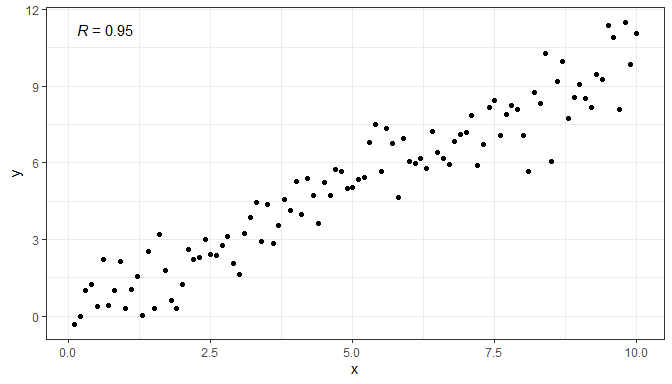Grouping is supported.

ggplot(my.data, aes(x, y, color = group)) +
geom_point() +
stat_correlation()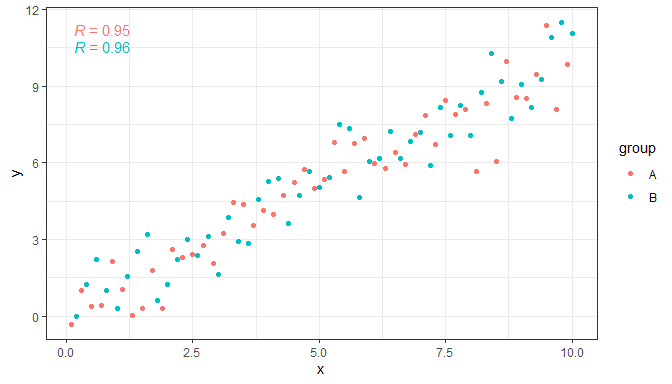We can also compute Spearman’s rank correlation. (The symbol used for it is the letter rho to distinguish it from Pearson’s correlation for which R or r are used as symbols.)

ggplot(my.data, aes(x, y, color = group)) +
geom_point() +
stat_correlation(method = "spearman")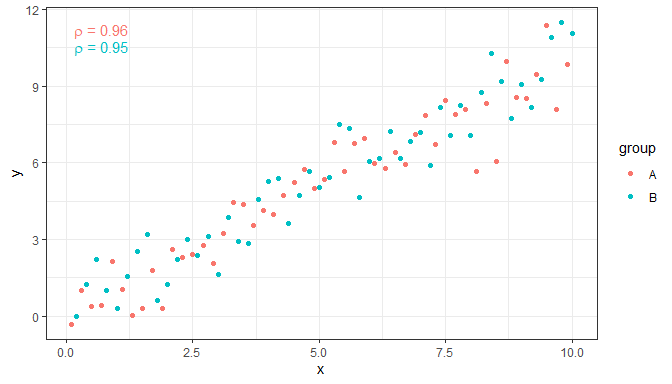Statistic stat_correlation() generates multiple labels as listed in the tables above. We can combine them freely within a call to aes() to customize the annotations.

ggplot(my.data, aes(x, y, color = group)) +
geom_point() +
stat_correlation(mapping = use_label(c("R", "t", "P", "n")))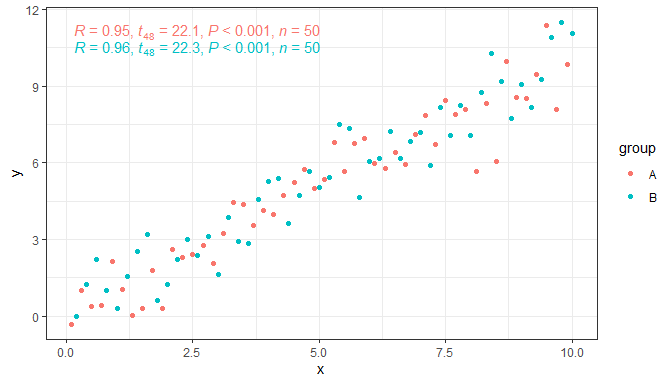Facets are also supported.

ggplot(my.data, aes(x, y)) +
geom_point() +
stat_correlation() +
facet_wrap(~group)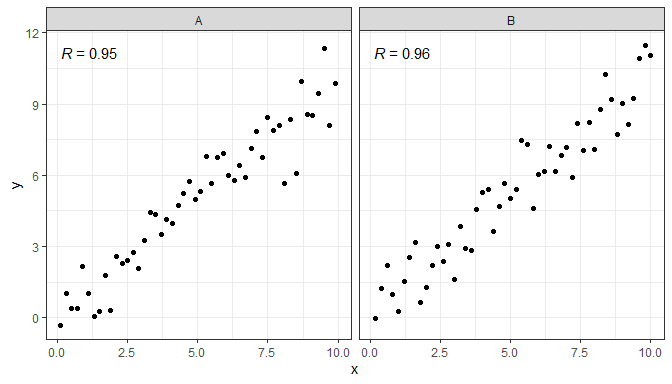Using the numeric values returned it is possible to set other aesthetics on-the-fly.

ggplot(my.data, aes(x, y)) +
geom_point() +
stat_correlation(mapping = aes(color = ifelse(after_stat(cor) > 0.955,
"red", "black"))) +
scale_color_identity() +
facet_wrap(~group)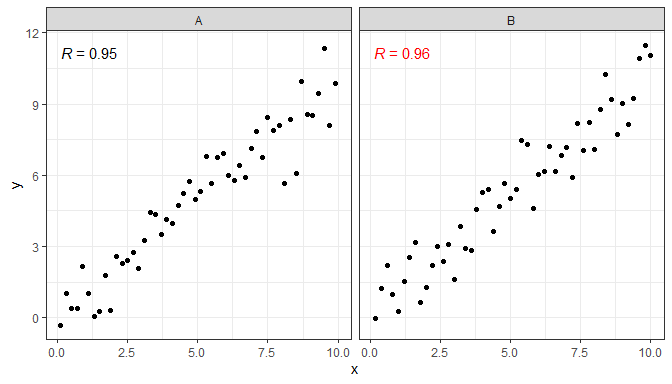Above we use the value of the correlation coefficient, but other numeric values such as p.value can be used similarly. In addition to colour, any aesthetics recognized by the geometry used such as alpha and face can be also mapped. The statistics described below for adding equations of the fitted models have user interfaces very similar to that of stat_correaltion() so examples can be easily adapted. Please, see the next section for examples of the use of different geometries instead of the default one, and other advanced examples.

### stat_poly_eq() and stat_poly_line()

A frequently used annotation in plots showing fitted lines is the display of the parameters estimates from the model fit as an equation. stat_poly_eq() automates this for models of y on x or x on y fitted with function lm(), and MASS:rlm() or a user-supplied model fitting function and arguments. By default this statistic behaves similarly as ggplot2::stat_smooth() with method = "lm" for y on x fits but adds support for model formulas of x on y (in addition to the use of orientation = "y"). Its default behaviour is to generate labels as character strings using R’s expression syntax. This statistic can also output equations using different mark-up languages, including $$\LaTeX$$ and Markdown, or just numeric values selected by the argument passed to parameter output.type. Nevertheless, all examples below use R’s expression syntax.

The statistic stat_poly_line() adds a layer nearly identical to that from ggplot2::stat_smooth() but has the same user interface and default arguments as stat_poly_eq().

We first generate a set of artificial data suitable for the plotting examples.

set.seed(4321)
# generate artificial data
x <- 1:100
y <- (x + x^2 + x^3) + rnorm(length(x), mean = 0, sd = mean(x^3) / 4)
y <- y / max(y)
my.data <- data.frame(x,
y,
group = c("A", "B"),
y2 = y * c(1, 2) + c(0, 0.2),
block = c("a", "a", "b", "b"),
wt = sqrt(x))

First, one example using defaults except for the model formula. The best practice, ensuring that the formula used in both statistics is the same is to assign the formula to a variable, as shown here. This is because the same model is fit twice to the same data, once in stat_smooth and once in stat_poly_eq.

We can write the model formula for a polynomial explicitly or using function poly(). When using function poly() it is in most cases necessary to set raw = TRUE as the default is to use orthogonal polynomials which although fitting the same line will have different estimates for the coefficients than those in the usual raw polynomials.

formula <- y ~ poly(x, 3, raw = TRUE)
ggplot(my.data, aes(x, y)) +
geom_point() +
stat_poly_line(formula = formula) +
stat_poly_eq(formula = formula)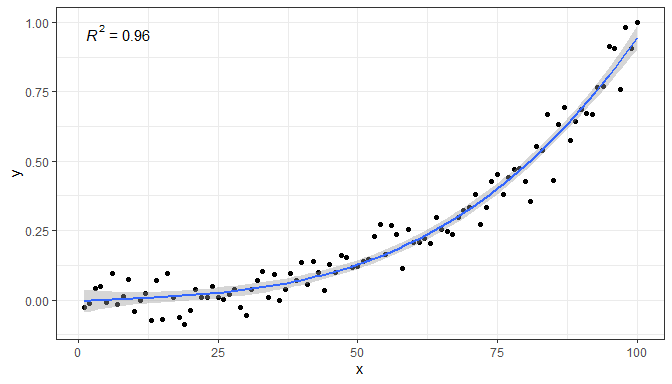A ready formatted equation is also returned as a string that needs to be parsed into an expression for display. Function use_label() can be used to select or assemble the expression and map it to the label aesthetic. The default separator is suitable for labels to be parsed into R expressions, but this default can be over-ridden by passing an argument to parameter sep. In this example we select the fitted model equation using key "eq" as argument to use_label() .

formula <- y ~ poly(x, 3, raw = TRUE)
ggplot(my.data, aes(x, y)) +
geom_point() +
stat_poly_line(formula = formula) +
stat_poly_eq(mapping = use_label("eq"), formula = formula)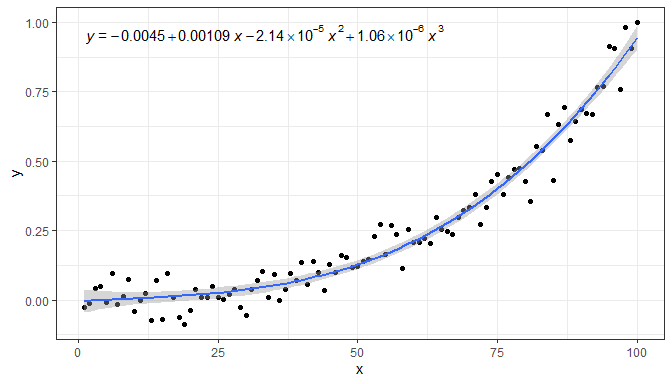The mapping can also be done with aes() using the name of the variable in the returned data containing the desired label and enclosing it in after_stat() to signal that it is a variable returned by the statistic.

formula <- y ~ poly(x, 3, raw = TRUE)
ggplot(my.data, aes(x, y)) +
geom_point() +
stat_poly_line(formula = formula) +
stat_poly_eq(mapping = aes(label = after_stat(eq.label)),
formula = formula)stat_poly_eq() makes available several character strings in the returned data frame in separate columns: eq.label, rr.label, adj.rr.label, AIC.label, BIC.label, f.value.label, p.value.label and n. One of these, rr.label, is used by default, but use_label() and aes() together with after_stat() can be used to map a different one to the label aesthetic. Here we show the adjusted coefficient of determination, $$R^2_\mathrm{adj}$$, Akaike’s information criterion, AIC. We call stat_poly_eq() twice to be able to separately control the position and size of each label. As parameter mapping is the first one in the statistics, we can pass the mapping by position (without writing mapping =).

formula <- y ~ poly(x, 3, raw = TRUE)
ggplot(my.data, aes(x, y)) +
geom_point() +
stat_poly_line(formula = formula) +
stat_poly_eq(mapping = use_label("adj.R2"), formula = formula) +
stat_poly_eq(mapping = use_label("AIC"), label.x = "right", label.y = "bottom", size = 3,
formula = formula)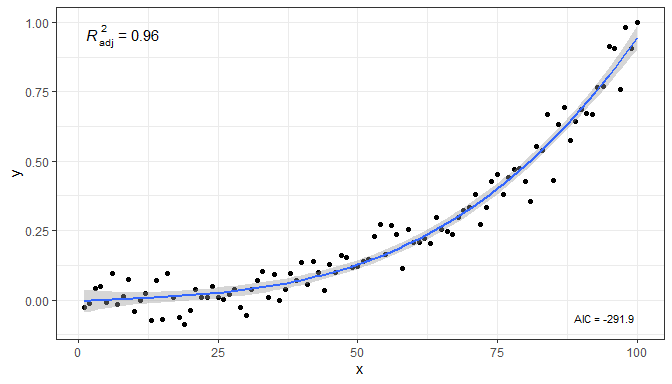Within aes() it is also possible to compute new labels based on those returned plus “arbitrary” text. The labels returned by default are meant to be parsed into expressions, so any text added should be valid for a string that will be parsed. Inserting a comma plus white space in an expression requires some trickery in the argument passed to parameter sep of paste(), or use_label(). Do note the need to escape the embedded quotation marks as \". Combining the labels in this way ensures correct alignment. To insert only white space sep = "~~~~~" can be used, with each tilde character, ~, adding a rather small amount of white space. We show here how to change the axis labels to ensure consistency with the typesetting of the equation.

formula <- y ~ poly(x, 3, raw = TRUE)
ggplot(my.data, aes(x, y)) +
geom_point() +
stat_poly_line(formula = formula) +
stat_poly_eq(mapping = use_label(c("eq", "adj.R2")),
formula = formula) +
labs(x = expression(italic(x)), y = expression(italic(y)))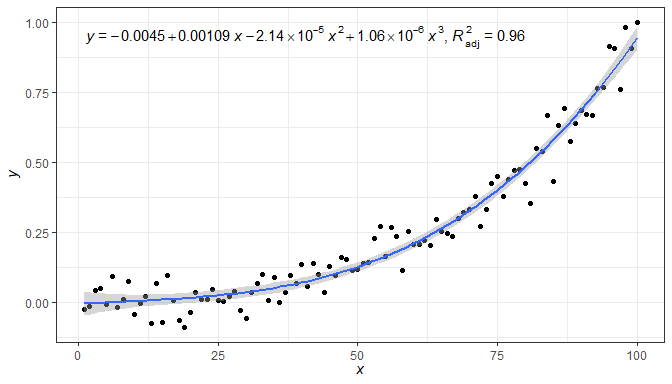Above we combined two character-string labels, but it is possible to add additional ones and even character strings constants. In the next example we use several labels instead of just two, and separate them with various character strings. In this case we need to use paste() and aes() as use_label() uses a single value for sep. We also change the size of the text in the label.

formula <- y ~ poly(x, 3, raw = TRUE)
ggplot(my.data, aes(x, y)) +
geom_point() +
stat_poly_line(formula = formula) +
stat_poly_eq(aes(label =  paste(after_stat(eq.label), "*\" with \"*",
after_stat(rr.label), "*\", \"*",
after_stat(f.value.label), "*\", and \"*",
after_stat(p.value.label), "*\".\"",
sep = "")),
formula = formula, size = 3)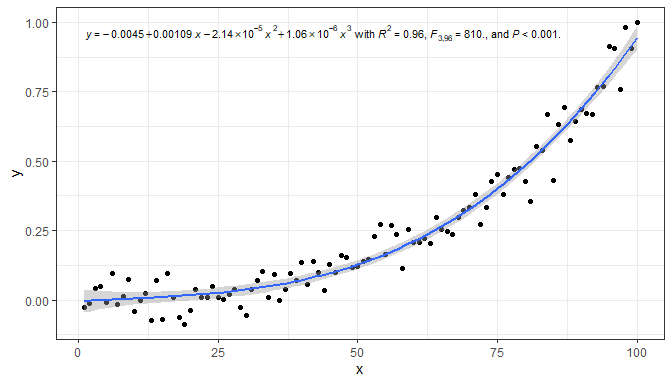It is also possible to format the text using plain(), italic(), bold() or bolditalic() an other commands as described in the help entry for plotmath.

formula <- y ~ poly(x, 3, raw = TRUE)
ggplot(my.data, aes(x, y)) +
geom_point() +
stat_poly_line(formula = formula) +
stat_poly_eq(mapping = use_label(c("eq", "adj.R2"), sep = "~~italic(\"with\")~~"),
formula = formula)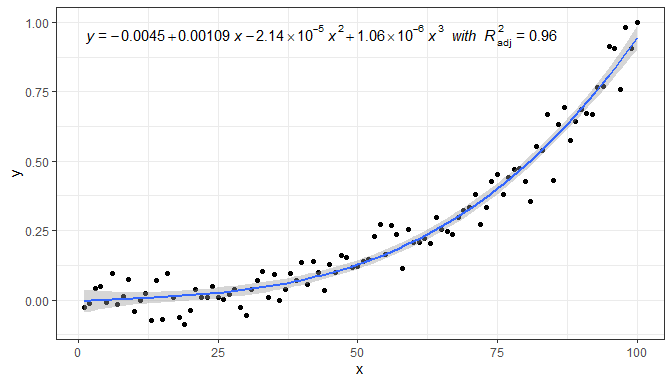As these are expressions, to include two lines of text, we need either to add stat_poly_eq() twice, passing an argument to label.y to reposition one of the labels (as shown above) or use (as shown here) atop() within the expression to create a single label with two lines of text.

formula <- y ~ poly(x, 3, raw = TRUE)
ggplot(my.data, aes(x, y)) +
geom_point() +
stat_poly_line(formula = formula) +
stat_poly_eq(aes(label = paste("atop(", after_stat(AIC.label), ",", after_stat(BIC.label), ")", sep = "")),
formula = formula)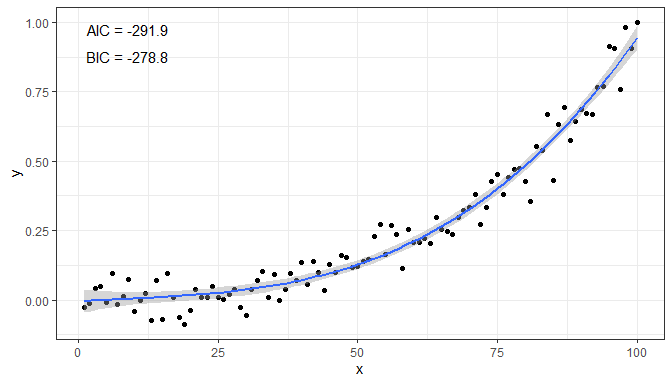Next, one example of how to remove the left hand side (lhs).

formula <- y ~ poly(x, 3, raw = TRUE)
ggplot(my.data, aes(x, y)) +
geom_point() +
stat_poly_line(formula = formula) +
stat_poly_eq(mapping = use_label("eq"),
eq.with.lhs = FALSE,
formula = formula)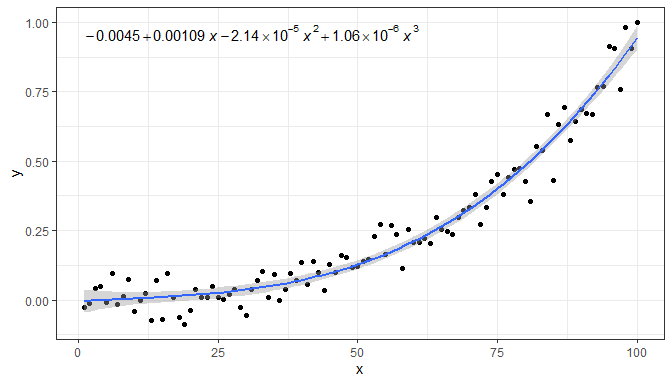Replacing the lhs.

formula <- y ~ poly(x, 3, raw = TRUE)
ggplot(my.data, aes(x, y)) +
geom_point() +
stat_poly_line(formula = formula) +
stat_poly_eq(mapping = use_label("eq"),
eq.with.lhs = "italic(hat(y))~=~",
formula = formula)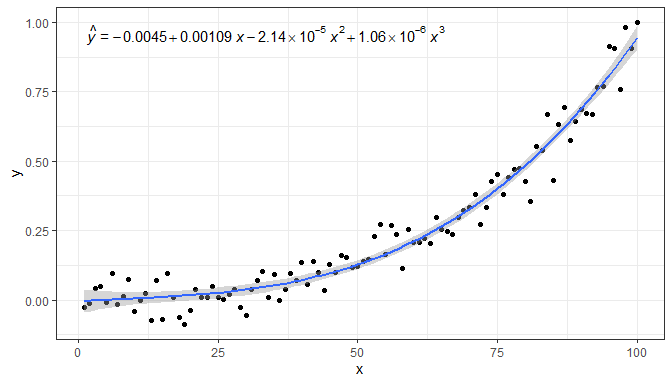Replacing both the lhs and the variable symbol used on the rhs.

formula <- y ~ poly(x, 3, raw = TRUE)
ggplot(my.data, aes(x, y)) +
geom_point() +
stat_poly_line(formula = formula) +
stat_poly_eq(mapping = use_label(c("eq", "R2")),
eq.with.lhs = "italic(h)~=~",
eq.x.rhs = "~italic(z)",
formula = formula) +
labs(x = expression(italic(z)), y = expression(italic(h)))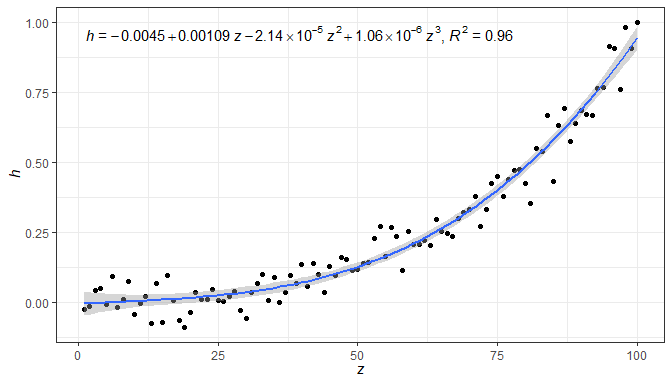As any valid R expression can be used, Greek letters are also supported, as well as the inclusion in the label of variable transformations used in the model formula or applied to the data. In addition, in the next example we insert white space in between the parameter estimates and the variable symbol in the equation.

formula <- y ~ poly(x, 2, raw = TRUE)
ggplot(my.data, aes(x, log10(y + 1e6))) +
geom_point() +
stat_poly_line(formula = formula) +
stat_poly_eq(mapping = use_label("eq"),
eq.with.lhs = "plain(log)(italic(delta)+10^6)~=~",
eq.x.rhs = "~Omega",
formula = formula) +
labs(y = expression(plain(log)(italic(delta)+10^6)), x = expression(Omega))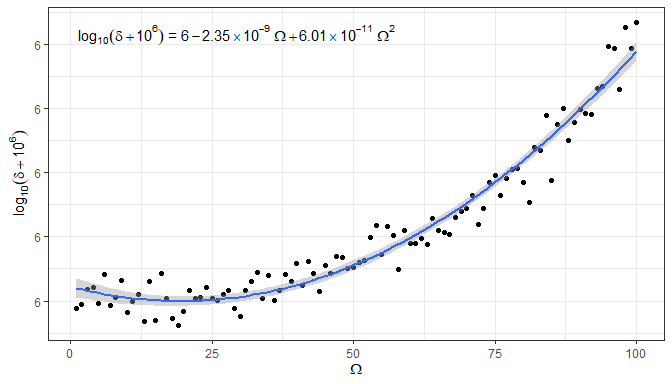Examples of polynomials of different orders, and fits through the origin. A degree 1 polynomial is linear regression with formula = y ~ x, which is the default. Linear regression through the origin is supported by passing formula = y ~ x - 1, or formula = y ~ x + 0. High order polynomials are most easily requested using poly() in the model formula, as shown next.

formula <- y ~ poly(x, 5, raw = TRUE)
ggplot(my.data, aes(x, y)) +
geom_point() +
stat_poly_line(formula = formula) +
stat_poly_eq(mapping = use_label("eq"), formula = formula)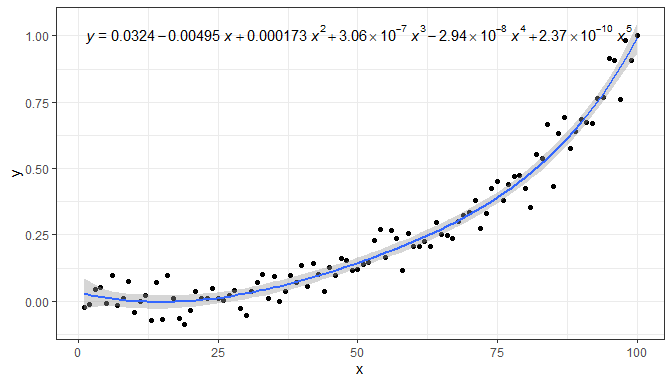Intercept forced to zero for a third degree polynomial defined explicitly, as this case is not supported by poly().

formula <- y ~ x + I(x^2) + I(x^3) - 1
ggplot(my.data, aes(x, y)) +
geom_point() +
stat_poly_line(formula = formula) +
stat_poly_eq(aes(label = after_stat(eq.label)), formula = formula)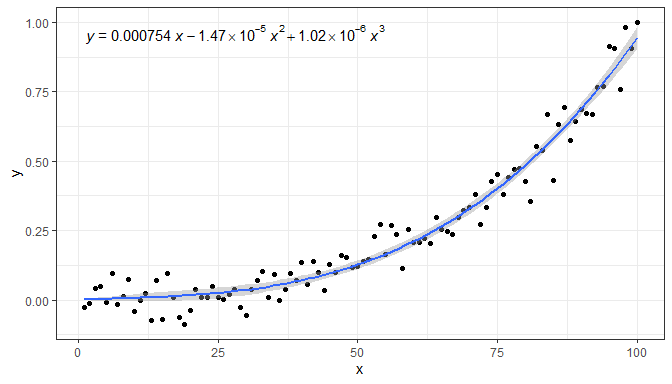Even when labels are returned, numeric values are also returned for r.squared, adj.r.squared, p.value and n. This can be used in aes() for example to show the equation only if a certain condition like a minimum value for r.square or p.value has been reached.

formula <- y ~ poly(x, 3, raw = TRUE)
ggplot(my.data, aes(x, y)) +
geom_point() +
stat_poly_line(formula = formula) +
stat_poly_eq(aes(label =  ifelse(after_stat(adj.r.squared > 0.3),
sep = "*\", \"*"),
formula = formula) +
labs(x = expression(italic(x)), y = expression(italic(y)))Fitting different models to different panels is supported. User-defined method functions are required to return an object that inherits from class "lm". The function is applied per group and panel, and the model formula fitted can differ among them, as it is retrieved from this object to construct the equation label.

In the example below instead of using lm() as method, we define a wrapper function that tests for the significance of the slope in linear regression, and if not significant, fits the mean instead.

poly_or_mean <- function(formula, data, ...) {
mf <- lm(formula = formula, data = data, ...)
if (anova(mf)[["Pr(>F)"]] > 0.1) {
lm(formula = y ~ 1, data = data, ...)
} else {
mf
}
}

We then use our function poly_or_mean() as the method.

ggplot(mpg, aes(displ, hwy)) +
geom_point() +
stat_poly_line(method = "poly_or_mean") +
stat_poly_eq(method = poly_or_mean,
aes(label = after_stat(eq.label)),
label.x = "right") +
theme(legend.position = "bottom") +
facet_wrap(~class, ncol = 2)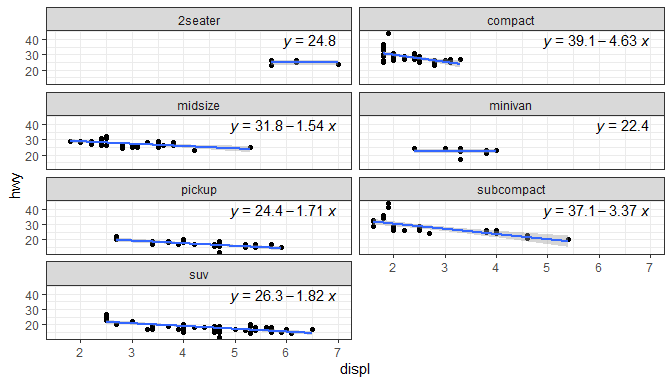We give below several examples to demonstrate how other components of the ggplot object affect the behaviour of this statistic.

Facets work as expected both with fixed or free scales. Although below we had to adjust the size of the font used for the equation.

formula <- y ~ poly(x, 3, raw = TRUE)
ggplot(my.data, aes(x, y2)) +
geom_point() +
stat_poly_line(formula = formula) +
stat_poly_eq(aes(label = after_stat(eq.label)), size = 3,
formula = formula) +
facet_wrap(~group)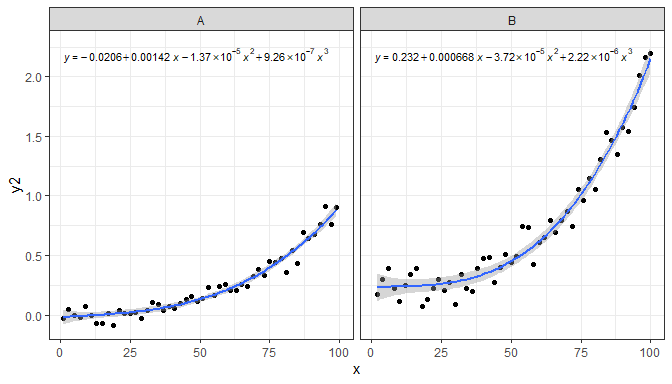formula <- y ~ poly(x, 3, raw = TRUE)
ggplot(my.data, aes(x, y2)) +
geom_point() +
stat_poly_line(formula = formula) +
stat_poly_eq(aes(label = after_stat(eq.label)), size = 3,
formula = formula) +
facet_wrap(~group, scales = "free_y")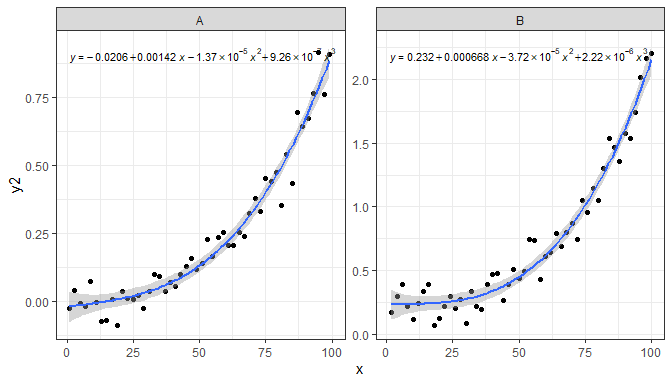Grouping works as expected. In this example we create groups using the colour aesthetic, but factors or descrete variables mapped to other aesthetics inlucing the “invisible” group aesthetic. We can use justification and supply an absolute location for the equation, but the default frequently works well as in the example below.

formula <- y ~ poly(x, 3, raw = TRUE)
ggplot(my.data, aes(x, y2, colour = group)) +
geom_point() +
stat_poly_line(formula = formula) +
stat_poly_eq(aes(label = after_stat(eq.label)), formula = formula, vstep = 0.06)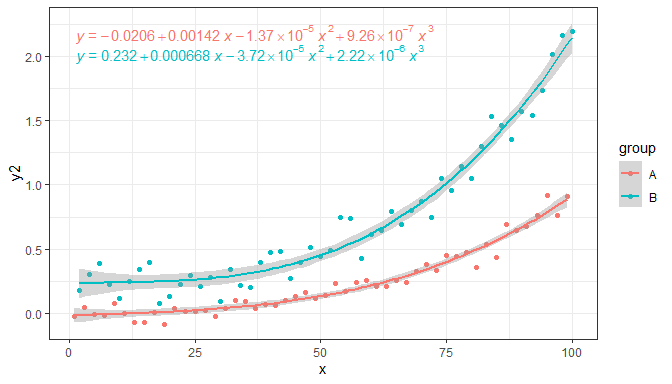To add a label to the equation for each group, we map it to a pseudo-aesthetic named grp.label.

formula <- y ~ poly(x, 3, raw = TRUE)
ggplot(my.data, aes(x, y2, colour = group, grp.label = group)) +
geom_point() +
stat_poly_line(formula = formula) +
stat_poly_eq(aes(label = after_stat(paste("bold(", grp.label, "*\":\")~~",
eq.label, sep = ""))),
formula = formula)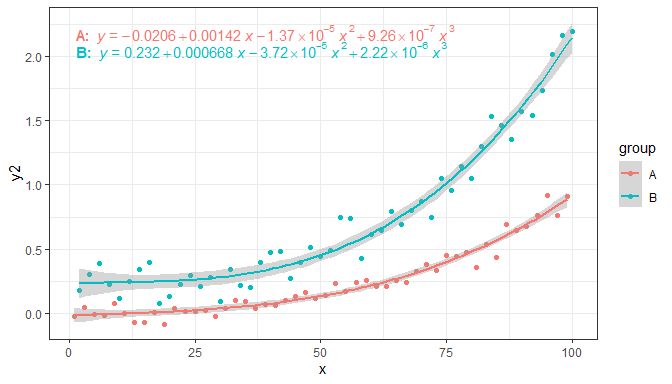Being able to label equations allows us to dispense with the use of colour, which in some cases is needed for publication in journals and books.

formula <- y ~ poly(x, 3, raw = TRUE)
ggplot(my.data, aes(x, y2, linetype = group, grp.label = group)) +
geom_point() +
stat_poly_line(formula = formula, color = "black") +
stat_poly_eq(aes(label = after_stat(paste("bold(", grp.label, "*':')~~~",
eq.label, sep = ""))),
formula = formula)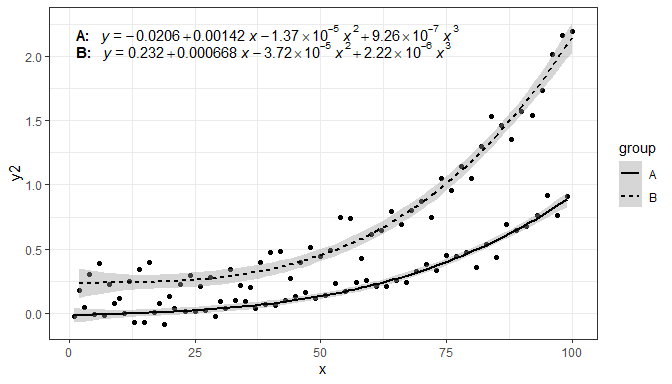User supplied label positions relative to the ranges of the x and y scales are also supported, both through string constants and numeric values in the range 0 to 1, when using the default geom_text_npc().

formula <- y ~ poly(x, 3, raw = TRUE)
ggplot(my.data, aes(x, y2, colour = group)) +
geom_point() +
stat_poly_line(formula = formula) +
stat_poly_eq(aes(label = after_stat(eq.label)),
formula = formula,
label.x = "centre")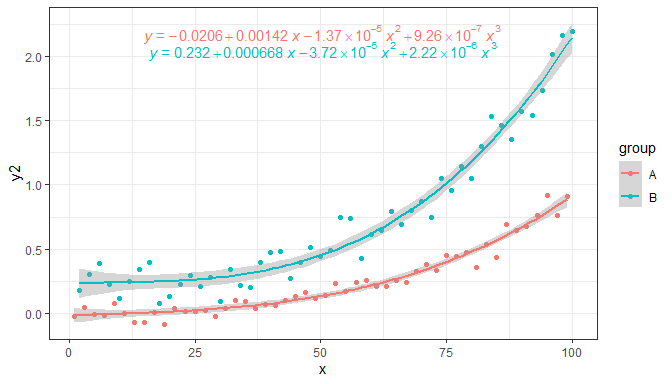The default locations are based on normalized coordinates, and consequently these defaults work even when the range of the x and y scales varies from panel to panel.

formula <- y ~ poly(x, 3, raw = TRUE)
ggplot(my.data, aes(x, y2, fill = block)) +
geom_point(shape = 21, size = 3) +
stat_poly_line(formula = formula) +
stat_poly_eq(aes(label = after_stat(rr.label)), size = 3,
geom = "label_npc", alpha = 0.33,
formula = formula) +
facet_wrap(~group, scales = "free_y")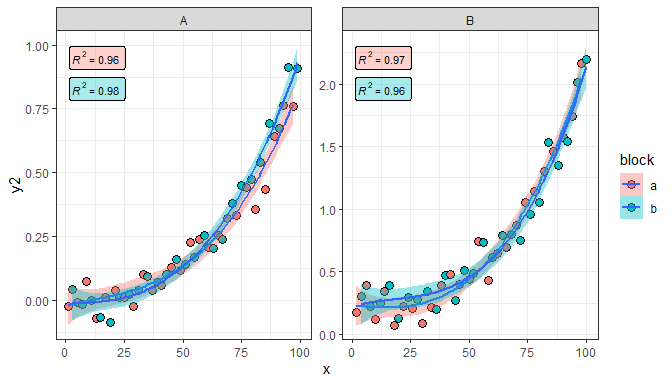If grouping is not the same within each panel created by faceting, i.e., not all groups have data in all panels, the automatic location of labels results in “holes” for the factor levels not present in a given panel. This behaviour ensures consistent positioning of labels for the same groups across panels. If positioning that leaves no gaps is desired, it is possible to explicitly pass as argument the positions for each individual label. In the plot below the simultaneous mapping to both colour and fill aesthetics creates four groups, two with data in panel A and the other two in panel B, but the vector passed as argument to label.y determines the y position (in npc units) four each of the four labels.

formula <- y ~ poly(x, 3, raw = TRUE)
ggplot(my.data, aes(x, y2, colour = group, fill = block)) +
geom_point(shape = 21, size = 3) +
stat_poly_line(formula = formula) +
stat_poly_eq(aes(label = after_stat(rr.label)), size = 3, alpha = 0.2,
geom = "label_npc", label.y = c(0.95, 0.85, 0.95, 0.85),
formula = formula) +
facet_wrap(~group, scales = "free_y")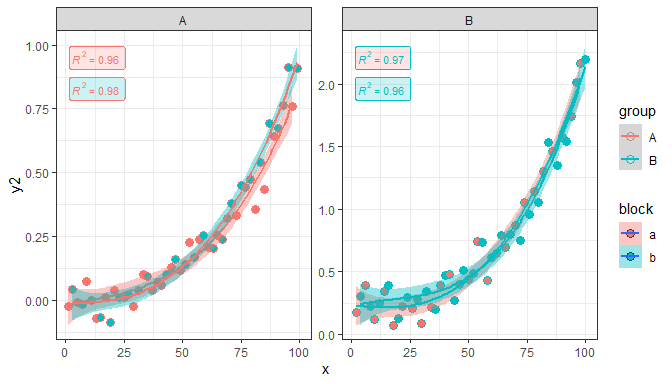It is possible to use geom_text() and geom_label() instead of the default geom_text_npc() (or geom_label_npc()) but in this case, if label coordinates are given explicitly they should be expressed in native data coordinates. When multiple labels need to be positioned a vector of coordinates can be used as shown here for label.x and label.y.

formula <- y ~ poly(x, 3, raw = TRUE)
ggplot(my.data, aes(x, y2, colour = group)) +
geom_point() +
stat_poly_line(formula = formula) +
stat_poly_eq(geom = "text", aes(label = after_stat(eq.label)),
label.x = c(100, 20), label.y = c(-0.1, 2.1), hjust = "inward",
formula = formula)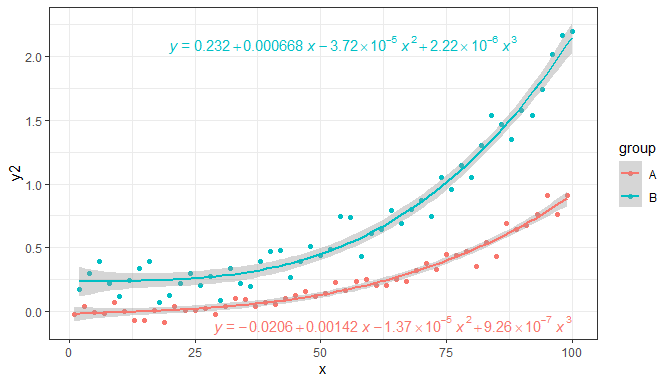Note: Automatic positioning using geom_text() and geom_label() is supported except when faceting uses free scales. In this case geom_text_npc() and/or geom_label_npc() or positions supplied by the user must be used.

Occasionally, possibly as a demonstration in teaching, we may want to compare a fit of x on y and one of y on x in the same plot. Here, this example also serves as introduction to the next section, which describes the use of major axis regression or Model II regression.

As is the case for model fitting functions themselves, which variable is dependent (reponse) and which is independent (explanatory) is simply indicated by the model equation passed as argument to formula, or consistently with stat_smooth() through parameter orientation as show below.

ggplot(my.data, aes(x, y)) +
geom_point() +
stat_poly_line(formula = y ~ x, color = "blue") +
stat_poly_eq(mapping = use_label(c("R2", "eq")), color = "blue") +
stat_poly_line(formula = y ~ x, color = "red", orientation = "y") +
stat_poly_eq(mapping = use_label(c("R2", "eq")), color = "red", orientation = "y",
label.y = 0.9)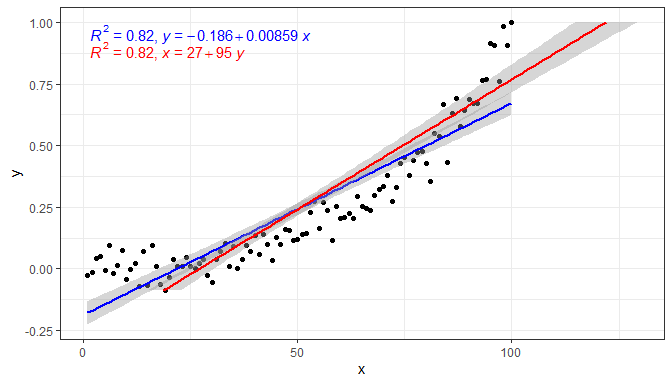### stat_ma_eq() and stat_ma_line()

Package ‘lmodel2’ implements Model II fits for major axis (MA), standard major axis (SMA) and ranged major axis (RMA) regression for linear relationships. These approaches of regression are used when both variables are subject to random variation and the intention is not to estimate y from x but instead to describe the slope of the relationship between them. A typical example in biology are allometric relationships. Rather frequently, OLS linear model fits are used in cases where Model II regression would be preferable.

In the figure above we see that the ordinary least squares (OLS) linear regression of y on x and x on y differ. Major axis regression provides an estimate of the slope that is independent of the direction of the relationship relation. $$R^2$$ remains the same in all cases, and with major axis regression while the coefficient estimates in the equation change, they correspond to exactly the same line.

ggplot(my.data, aes(x, y)) +
geom_point() +
stat_ma_line() +
stat_ma_eq(mapping = use_label("eq"))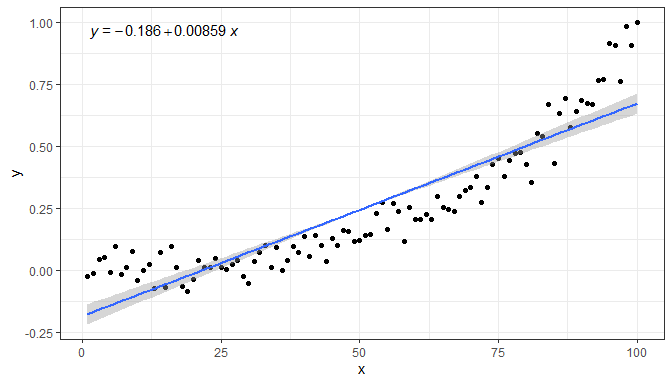ggplot(my.data, aes(x, y)) +
geom_point() +
stat_ma_line(color = "blue") +
stat_ma_eq(mapping = use_label(c("R2", "eq")), color = "blue") +
stat_ma_line(color = "red", orientation = "y") +
stat_ma_eq(mapping = use_label(c("R2", "eq")), color = "red", orientation = "y",
label.y = 0.9)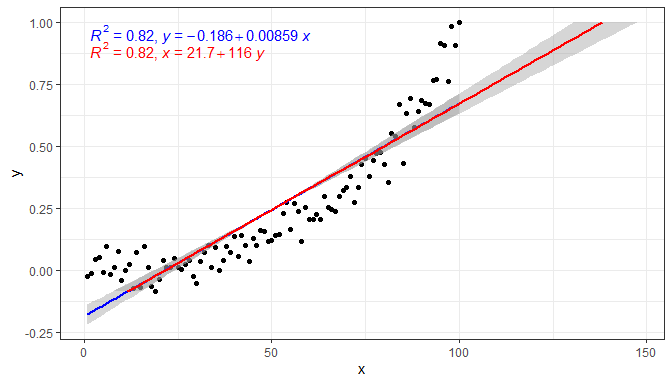### stat_quant_eq(), stat_quant_line() and stat_quant_band()

Package ‘quantreg’ implements quantile regression for multiple types of models: linear models with function rq(), non-linear models with function nlreg(), and additive models with function rqss(). Each of these functions supports different methods of estimation which can be passed through parameter method.args or more easily using a character string such as "rq:br" argument to parameter method of the statistic, where rq() is the model fit function and "br" the argument passed to the method parameter of rq(). As with the statistics for polynomials described in the previous section, all the statistics described in the current section support linear models while stat_quant_line() and stat_quant_band() also support additive models. (Support for nlreg() is not yet implemented.)

In the case of quantile regression it is frequently the case that multiple quantile regressions are fitted. This case is not supported by stat_poly_eq() and we need to use stat_quant_eq(). This statistics is very similar to stat_poly_eq() both in behaviour and parameters. It supports quantile regression of y on x or x on y fitted with function quantreg::rq.

The statistic stat_quant_line() adds a layer similar to that created by ggplot2::stat_smooth() including confidence bands for quantile regression, by default for p = 0.95. However, it has the same user interface and default arguments as stat_quant_eq(), and supports both x and y as explanatory variable in formula and also orientation. Confidence intervals for the fits to individual quantiles fulfil the same purpose as in OLS fits: providing a boundary for the estimated line computed for each individual quantile. They are not shown by default, unless a single quantile is passed as argument, but can be enabled with se = TRUE and disabled with se = FALSE.

The statistic stat_quant_band() creates a line plus band plot that is, by default equivalent to the box of a box plot showing regressions as a band for quartiles plus a line for the median regression. In this case, the band informs about the spread of the observations in the same way as the box in box plots does. Which three quantiles are fitted can be set through parameter quantiles but in contrast to stat_quant_line() and stat_quant_eq() only a vector of two or three quantiles is accepted (with two quantiles the line is omitted and only a band displayed).

Similarly to ggplot2::stat_quantiles(), stat_quant_eq() and stat_quant_line() accept a numeric vector as argument to quantiles and output one equation or one line per group and quantile. Statistic stat_quant_eq() also returns column grp.label containing by default a character string indicating the value of the quantile, and if a variable is mapped to the pseudo aesthetic grp.label its value is prepended to the values of the quantiles.

Here we use the default value for quantiles, c(0.25, 0.50, 0.75), equivalent to those used for the box of box plots, and we obtain a plot with a line for median regression and a band highlighting the space between the quartiles.

ggplot(my.data, aes(x, y)) +
geom_point() +
stat_quant_band(formula = y ~ poly(x, 2))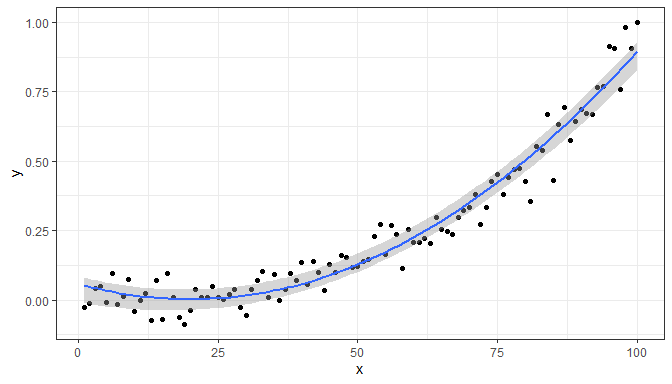Overriding the values for quantiles we find a boundary containing 90% of the observations.

ggplot(my.data, aes(x, y)) +
geom_point() +
stat_quant_line(formula = y ~ poly(x, 2), quantiles = c(0.05, 0.95))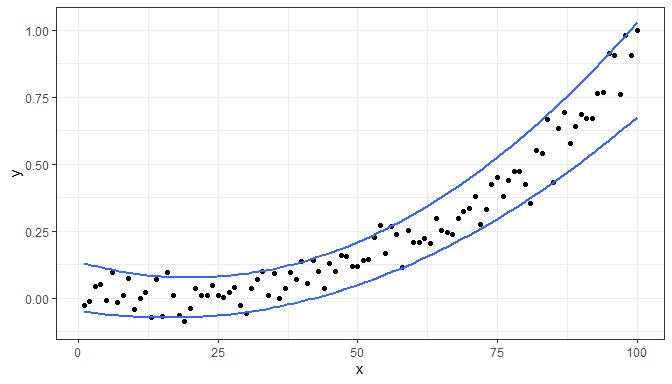A line for the quantile regression plus a confidence band for it is the default for a single quantile. (This is similar to the behaviour of ggplot2::stat_smooth().)

ggplot(my.data, aes(x, y)) +
geom_point() +
stat_quant_line(formula = y ~ poly(x, 2), quantiles = 0.5)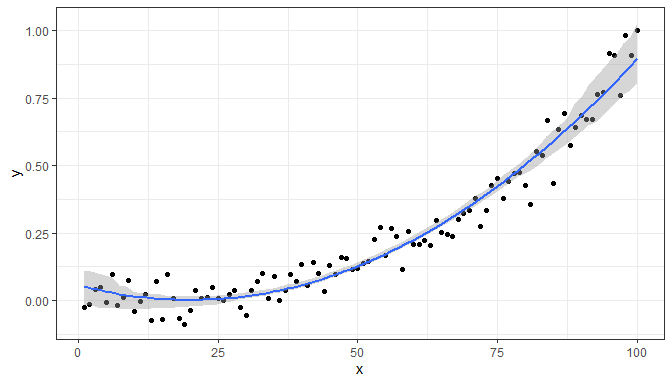With stat_quant_eq() we add the fitted equations to the first example in this section (we also override the use of colour).

ggplot(my.data, aes(x, y)) +
geom_point() +
stat_quant_band(formula = formula, color = "black", fill = "grey60") +
stat_quant_eq(aes(label = paste(after_stat(grp.label), "*\": \"*",
after_stat(eq.label), sep = "")),
formula = formula) +
theme_classic()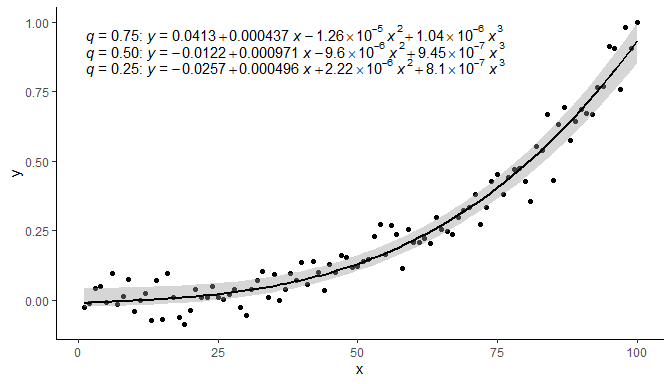Grouping and faceting are supported by stat_quant_eq(), stat_quant_line() and stat_quant_band(). Below are some examples of grouping.

ggplot(my.data, aes(x, y, color = group)) +
geom_point() +
stat_quant_line(formula = formula) +
stat_quant_eq(aes(label = paste(after_stat(grp.label), "*\": \"*",
after_stat(eq.label), sep = "")),
formula = formula)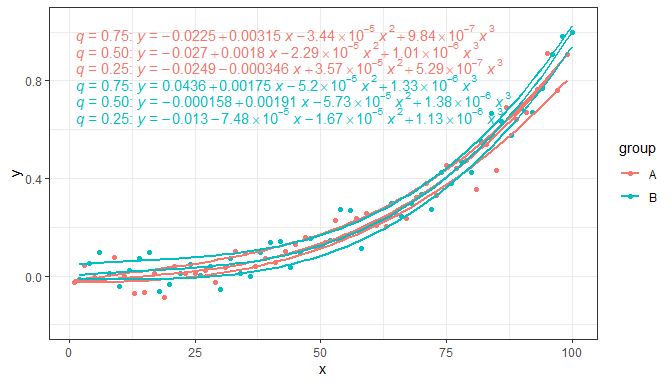Grouping can be combined with a group label by mapping in aes() a character variable to grp.label.

ggplot(my.data, aes(x, y, group = group, linetype = group,
shape = group, grp.label = group)) +
geom_point() +
stat_quantile(formula = formula, color = "black") +
stat_quant_eq(aes(label = paste(after_stat(grp.label), "*\": \"*",
after_stat(eq.label), sep = "")),
formula = formula, quantiles = c(0.05, 0.95)) +
theme_classic()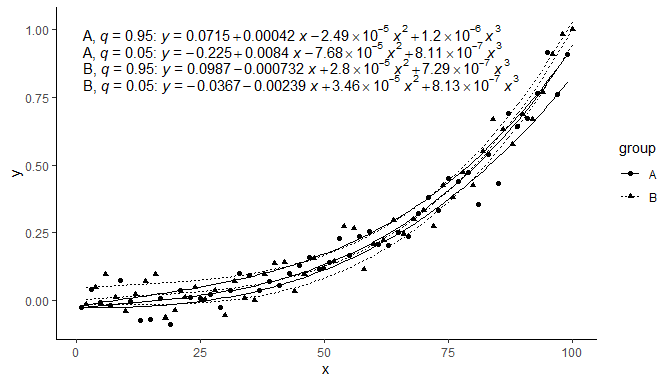In some cases double quantile regression is an informative method to assess reciprocal constraints. For this a fit of x on y and one of y on x in the same plot are computed for a large quantile. As is the case for model fitting functions themselves this is simply indicated by the model passed as argument to formula, or consistently with ggplot2::stat_smooth() through parameter orientation.

ggplot(my.data, aes(x, y)) +
geom_point() +
stat_quant_line(formula = y ~ x, color = "blue", quantiles = 0.05) +
stat_quant_eq(mapping = use_label("eq"), formula = y ~ x, color = "blue",
quantiles = 0.05) +
stat_quant_line(formula = x ~ y, color = "red", quantiles = 0.95) +
stat_quant_eq(mapping = use_label("eq"), formula = x ~ y, color = "red",
quantiles = 0.95, label.y = 0.9)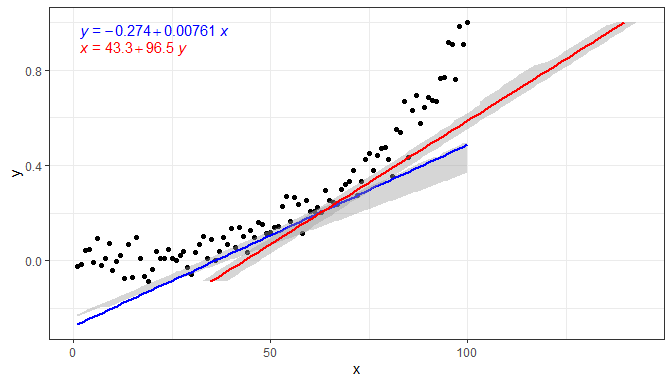Please, see the section on stat_poly_eq() for additional examples, as here we have highlighted mainly the differences between these two statistics.

### stat_fit_residuals

Sometimes we need to quickly plot residuals matching fits plotted with geom_poly_line(), geom_quant_line() or geom_smooth() using grouping and facets. stat_fit_residuals allows thia. In teaching it is specially important to avoid distractions and plots residuals from different types of models consistently.

stat_fit_residuals() can be used to plot the residuals from models of y on x or x on y fitted with function lm, MASS:rlm, quantreg::rq (only median regression) or a user-supplied model fitting function and arguments. In the returned values the response variable is replaced by the residuals from the fit. The default geom is "point".

formula <- y ~ poly(x, 3, raw = TRUE)
ggplot(my.data, aes(x, y, colour = group)) +
geom_hline(yintercept = 0, linetype = "dashed") +
stat_fit_residuals(formula = formula)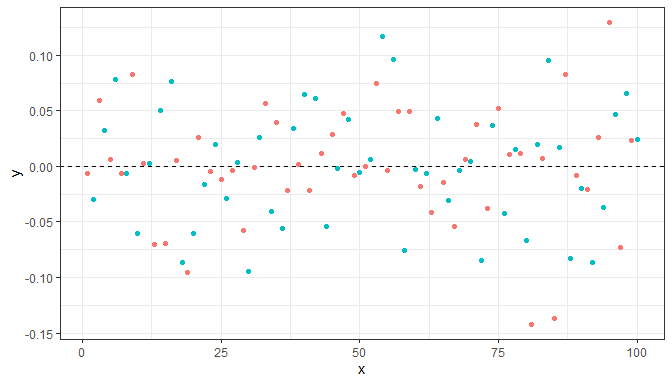We can of course also map the weights returned in the model fit object to ggplot2 aesthetics, here we use size.

formula <- y ~ poly(x, 3, raw = TRUE)
ggplot(my.data, aes(x, y, colour = group)) +
geom_hline(yintercept = 0, linetype = "dashed") +
stat_fit_residuals(formula = formula,
method = "rlm",
mapping = aes(size = sqrt(after_stat(weights))),
alpha = 2/3)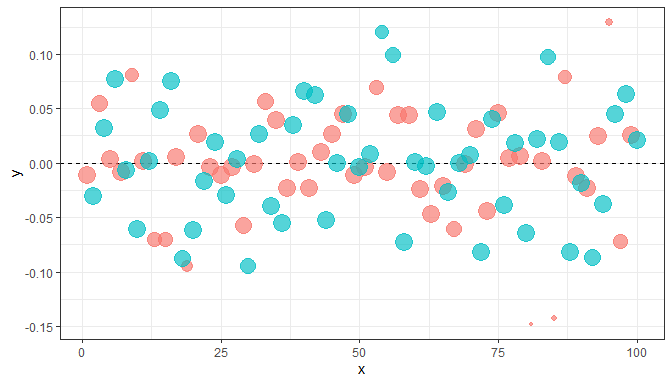Weighted residuals are available, but in this case they do not differ as we have not mapped any variable to the aesthetic.

formula <- y ~ poly(x, 3, raw = TRUE)
ggplot(my.data, aes(x, y, colour = group)) +
geom_hline(yintercept = 0, linetype = "dashed") +
stat_fit_residuals(formula = formula, weighted = TRUE)

### stat_fit_deviations

When teaching we may need to highlight residuals in plots used in slides and notes. Statistic stat_fit_deviations makes it easy to achieve this for the residuals from models of y on x or x on y fitted with function lm, MASS:rlm, quantreg::rq (only median regression) or a user-supplied model fitting function and arguments. The default geom is "segment" and the values of the the observations and the fitted values are mapped to aesthetics so that each deviation is displayed as a segment.

formula <- y ~ poly(x, 3, raw = TRUE)
ggplot(my.data, aes(x, y)) +
geom_smooth(method = "lm", formula = formula) +
stat_fit_deviations(formula = formula, colour = "red") +
geom_point()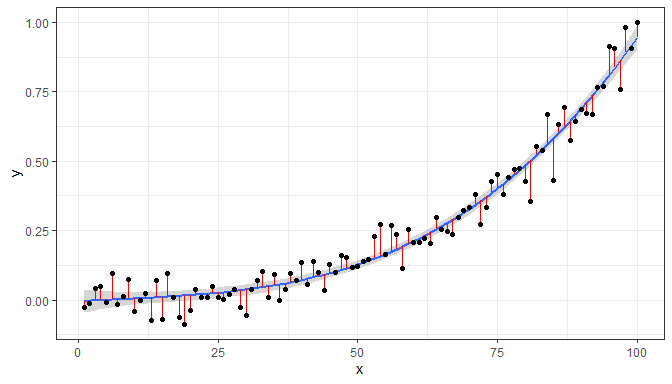As the geometry used by default is geom_segment(), additional aesthetics can be mapped or set to constant values. Here we add arrowheads.

formula <- y ~ poly(x, 3, raw = TRUE)
ggplot(my.data, aes(x, y)) +
geom_smooth(method = "lm", formula = formula) +
geom_point() +
stat_fit_deviations(formula = formula, colour = "red",
arrow = arrow(length = unit(0.015, "npc"),
ends = "both"))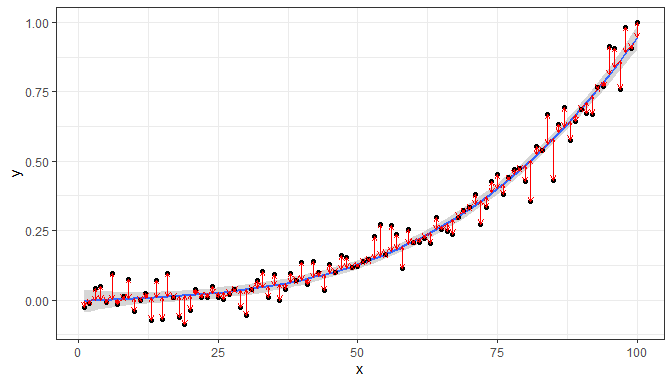When weights are available, either supplied by the user, or computed as part of the fit, they are returned in data. Having weights available allows encoding them using colour. We here use a robust regression fit with MASS::rlm.

my.data.outlier <- my.data
my.data.outlier[6, "y"] <- my.data.outlier[6, "y"] * 5
ggplot(my.data.outlier, aes(x, y)) +
stat_smooth(method = MASS::rlm, formula = formula) +
stat_fit_deviations(formula = formula, method = "rlm",
mapping = aes(colour = after_stat(weights)),
show.legend = TRUE) +
scale_color_gradient(low = "red", high = "blue", limits = c(0, 1)) +
geom_point()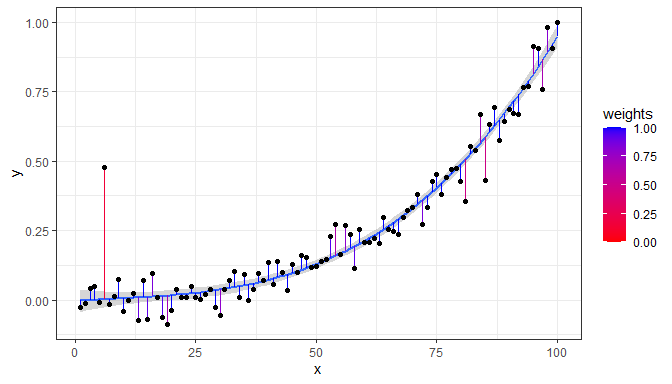### stat_fit_glance

Package ‘broom’ provides consistently formatted output from different model fitting functions. These made it possible to write a model-annotation statistic that is very flexible but that requires additional input from the user to generate the character strings to be mapped to the label aesthetic.

As we have above given some simple examples, we here exemplify this statistic in a plot with grouping, and assemble a label for the P-value using a string parsed into a expression. We also change the default position of the labels.

# formula <- y ~ poly(x, 3, raw = TRUE)
# broom::augment does not handle poly() correctly!
formula <- y ~ x + I(x^2) + I(x^3)
ggplot(my.data, aes(x, y, colour = group)) +
geom_point() +
geom_smooth(method = "lm", formula = formula) +
stat_fit_glance(method = "lm",
method.args = list(formula = formula),
label.x = "right",
label.y = "bottom",
aes(label = sprintf("italic(P)*\"-value = \"*%.3g",
after_stat(p.value))),
parse = TRUE)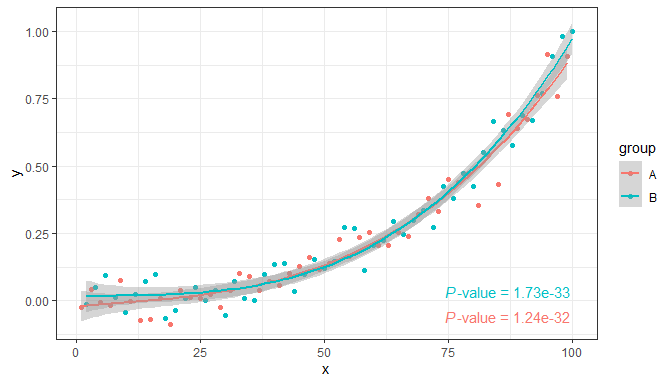It is also possible to fit a non-linear model with method = "nls", and any other model for which a glance() method exists. Do consult the documentation for package ‘broom’. Here we fit the Michaelis-Menten equation to reaction rate versus concentration data.

micmen.formula <- y ~ SSmicmen(x, Vm, K)
ggplot(Puromycin, aes(conc, rate, colour = state)) +
geom_point() +
geom_smooth(method = "nls",
formula = micmen.formula,
se = FALSE) +
stat_fit_glance(method = "nls",
method.args = list(formula = micmen.formula),
aes(label = paste("AIC = ", signif(after_stat(AIC), digits = 3),
", BIC = ", signif(after_stat(BIC), digits = 3),
sep = "")),
label.x = "centre", label.y = "bottom")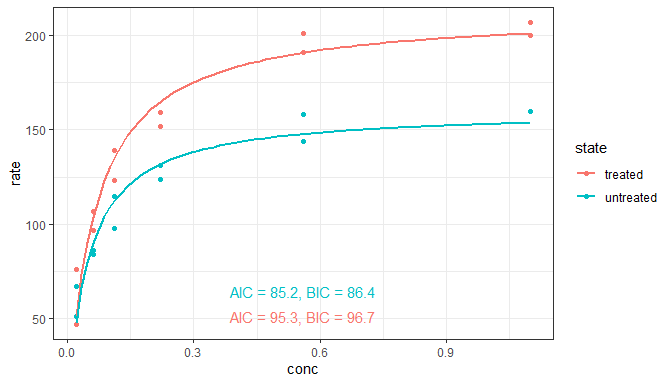### stat_fit_tb

This stat makes it possible to add summary or ANOVA tables for any fitted model for which broom::tidy() is implemented. The output from tidy() is embedded as a single list value within the returned data, an object of class tibble. This statistic ignores grouping based on aesthetics. This allows fitting models when x or y is a factor (as in such cases ggplot splits the data into groups, one for each level of the factor, which is needed for example for stat_summary() to work as expected). By default, the "table" geometry is used. The use of geom_table() is described in a separate section of this User Guide. Table themes and mapped aesthetics are supported.

The default output of stat_fit_tb is the default output from tidy(mf) where mf is the fitted model.

formula <- y ~ x + I(x^2) + I(x^3)
ggplot(my.data, aes(x, y)) +
geom_point() +
geom_smooth(method = "lm", formula = formula) +
stat_fit_tb(method = "lm",
method.args = list(formula = formula),
tb.vars = c(Parameter = "term",
Estimate = "estimate",
"s.e." = "std.error",
"italic(t)" = "statistic",
"italic(P)" = "p.value"),
label.y = "top", label.x = "left",
parse = TRUE)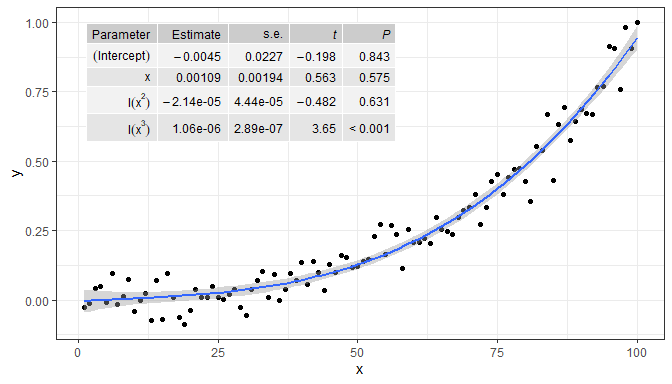When tb.type = "fit.anova" the output returned is that from tidy(anova(mf)) where mf is the fitted model. Here we also show how to replace names of columns and terms, and exclude one column, in this case, the mean squares.

formula <- y ~ x + I(x^2) + I(x^3)
ggplot(my.data, aes(x, y)) +
geom_point() +
geom_smooth(method = "lm", formula = formula) +
stat_fit_tb(method = "lm",
method.args = list(formula = formula),
tb.type = "fit.anova",
tb.vars = c(Effect = "term",
df = "df",
"italic(F)" = "statistic",
"italic(P)" = "p.value"),
tb.params = c(x = 1, "x^2" = 2, "x^3" = 3, Resid = 4),
label.y = "top", label.x = "left",
parse = TRUE)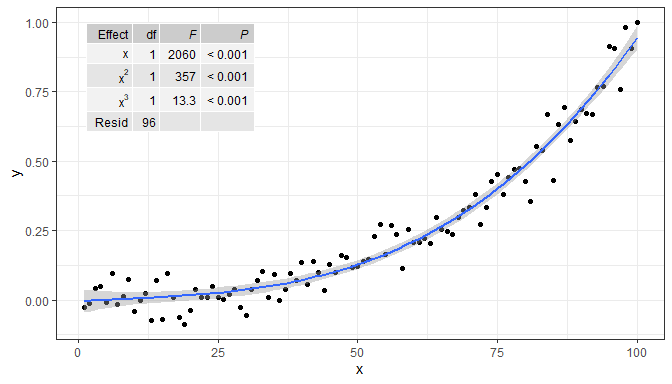When tb.type = "fit.coefs" the output returned is that of tidy(mf) after selecting the term and estimate columns.

formula <- y ~ x + I(x^2) + I(x^3)
ggplot(my.data, aes(x, y)) +
geom_point() +
geom_smooth(method = "lm", formula = formula) +
stat_fit_tb(method = "lm",
method.args = list(formula = formula),
tb.type = "fit.coefs", parse = TRUE,
label.y = "center", label.x = "left")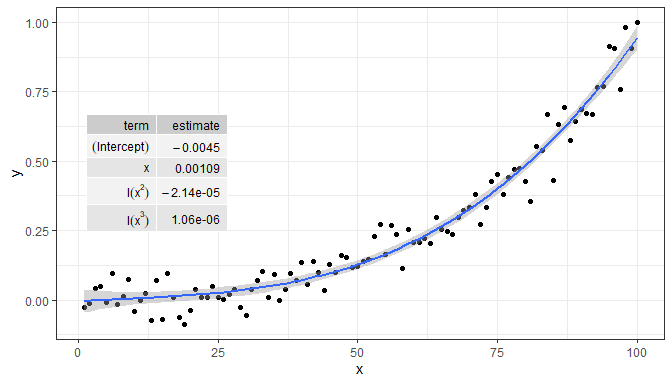Faceting works as expected, but grouping is ignored as mentioned above. In this case, the color aesthetic is not applied to the text of the tables. Furthermore, if label.x.npc or label.y.npc are passed numeric vectors of length > 1, the corresponding values are obeyed by the different panels.

micmen.formula <- y ~ SSmicmen(x, Vm, K)
ggplot(Puromycin, aes(conc, rate, colour = state)) +
facet_wrap(~state) +
geom_point() +
geom_smooth(method = "nls",
formula = micmen.formula,
se = FALSE) +
stat_fit_tb(method = "nls",
method.args = list(formula = micmen.formula),
tb.type = "fit.coefs",
label.x = 0.9,
label.y = c(0.75, 0.2)) +
theme(legend.position = "none") +
labs(x = "C", y = "V")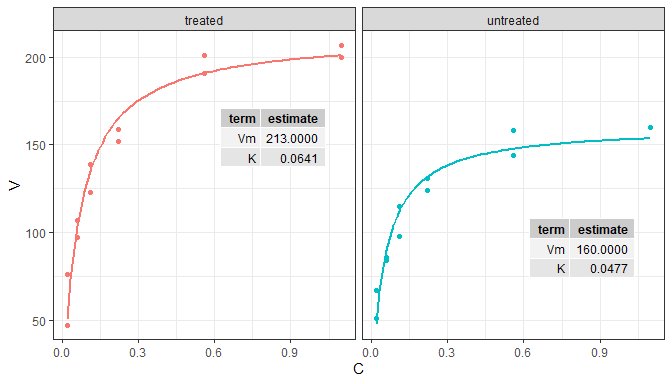The data in the example below are split by ggplot into six groups based on the levels of the feed factor. However, as stat_fit_tb() ignores groupings, we can still fit a linear model to all the data in the panel.

ggplot(chickwts, aes(factor(feed), weight)) +
stat_summary(fun.data = "mean_se") +
stat_fit_tb(tb.type = "fit.anova",
label.x = "center",
label.y = "bottom") +
expand_limits(y = 0)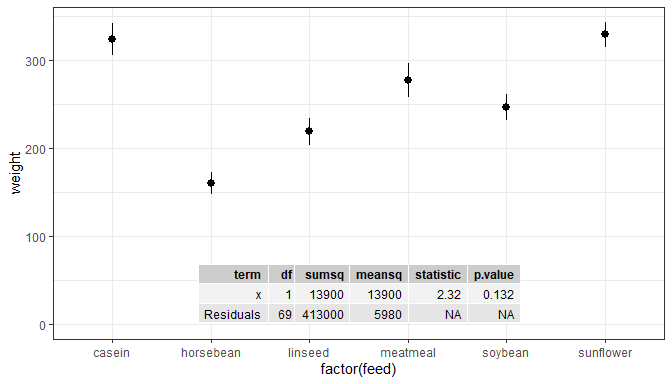We can flip the system of coordinates, if desired.

ggplot(chickwts, aes(factor(feed), weight)) +
stat_summary(fun.data = "mean_se") +
stat_fit_tb(tb.type = "fit.anova", label.x = "left", size = 3) +
scale_x_discrete(expand = expansion(mult = c(0.2, 0.5))) +
coord_flip()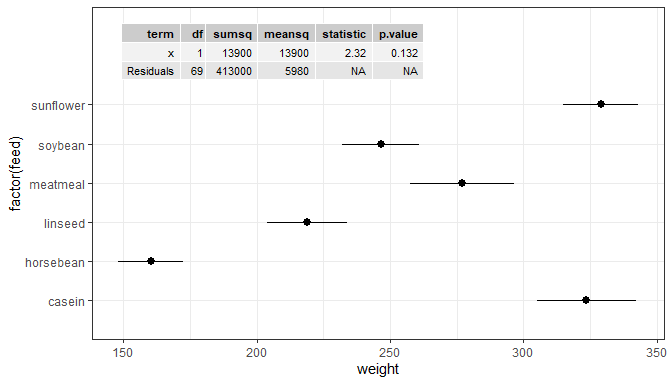It is also possible to rotate the table using angle. Here we also show how to replace the column headers with strings to be parsed into R expressions.

ggplot(chickwts, aes(factor(feed), weight)) +
stat_summary(fun.data = "mean_se") +
stat_fit_tb(tb.type = "fit.anova",
angle = 90, size = 3,
label.x = "right", label.y = "center",
hjust = 0.5, vjust = 0,
tb.vars = c(Effect = "term",
"df",
"M.S." = "meansq",
"italic(F)" = "statistic",
"italic(P)" = "p.value"),
parse = TRUE) +
scale_x_discrete(expand = expansion(mult = c(0.1, 0.35))) +
expand_limits(y = 0)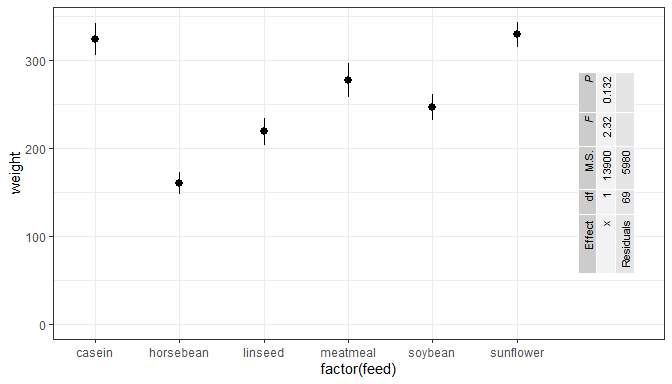### stat_fit_tidy

This stat makes it possible to add the equation for any fitted model for which broom::tidy() is implemented. Alternatively, individual values such as estimates for the fitted parameters, standard errors, or P-values can be added to a plot. However, the user has to explicitly construct the labels within aes(). This statistic respects grouping based on aesthetics, and reshapes the output of tidy() so that the values for a given group are in a single row in the returned data.

As first example we fit a non-linear model, the Michaelis-Menten equation of reaction kinetics, using nls(). We use the self-starting function SSmicmen() available in R.

micmen.formula <- y ~ SSmicmen(x, Vm, K)
ggplot(Puromycin, aes(conc, rate, colour = state)) +
geom_point() +
geom_smooth(method = "nls",
formula = micmen.formula,
se = FALSE) +
stat_fit_tidy(method = "nls",
method.args = list(formula = micmen.formula),
label.x = "right",
label.y = "bottom",
aes(label = paste("V[m]~=~", signif(after_stat(Vm_estimate), digits = 3),
"%+-%", signif(after_stat(Vm_se), digits = 2),
"~~~~K~=~", signif(after_stat(K_estimate), digits = 3),
"%+-%", signif(after_stat(K_se), digits = 2),
sep = "")),
parse = TRUE)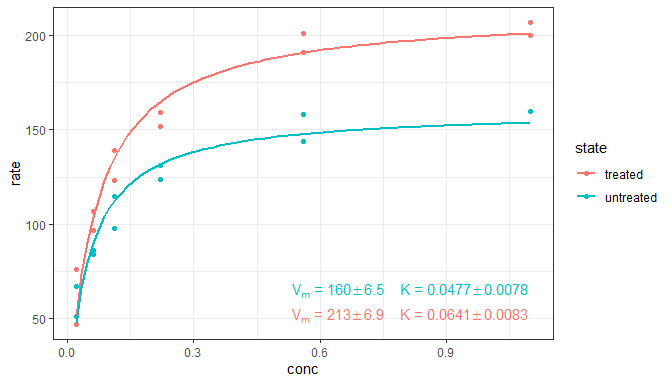Using paste we can build a string that can be parsed into an R expression, in this case for a non-linear equation.

micmen.formula <- y ~ SSmicmen(x, Vm, K)
ggplot(Puromycin, aes(conc, rate, colour = state)) +
geom_point() +
geom_smooth(method = "nls",
formula = micmen.formula,
se = FALSE) +
stat_fit_tidy(method = "nls",
method.args = list(formula = micmen.formula),
size = 3,
label.x = "center",
label.y = "bottom",
vstep = 0.12,
aes(label = paste("V~=~frac(", signif(after_stat(Vm_estimate), digits = 2), "~C,",
signif(after_stat(K_estimate), digits = 2), "+C)",
sep = "")),
parse = TRUE) +
labs(x = "C", y = "V")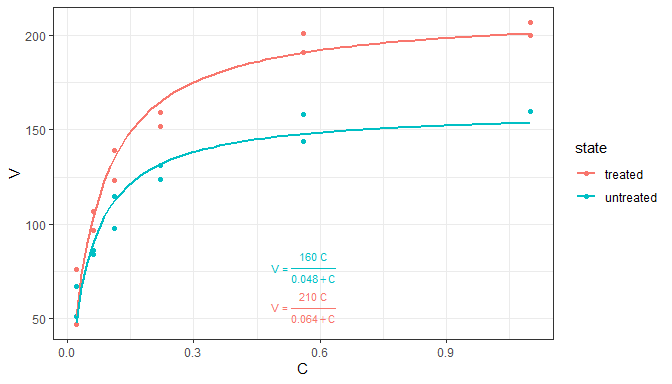What if we would need a more specific statistic, similar to stat_poly_eq()? We can use stat_fit_tidy() as the basis for its definition.

stat_micmen_eq <- function(vstep = 0.12,
size = 3,
...) {
stat_fit_tidy(method = "nls",
method.args = list(formula = micmen.formula),
aes(label = paste("V~=~frac(", signif(after_stat(Vm_estimate), digits = 2), "~C,",
signif(after_stat(K_estimate), digits = 2), "+C)",
sep = "")),
parse = TRUE,
vstep = vstep,
size = size,
...)
}

The code for the figure is now simpler, and still produces the same figure (not shown).

micmen.formula <- y ~ SSmicmen(x, Vm, K)
ggplot(Puromycin, aes(conc, rate, colour = state)) +
geom_point() +
geom_smooth(method = "nls",
formula = micmen.formula,
se = FALSE) +
stat_micmen_eq(label.x = "center",
label.y = "bottom") +
labs(x = "C", y = "V")• As a second example we show a quantile regression fit using function rq() from package ‘quantreg’.
my_formula <- y ~ x

ggplot(mpg, aes(displ, 1 / hwy)) +
geom_point() +
geom_quantile(quantiles = 0.5, formula = my_formula) +
stat_fit_tidy(method = "rq",
method.args = list(formula = y ~ x, tau = 0.5),
tidy.args = list(se.type = "nid"),
mapping = aes(label = sprintf('y~"="~%.3g+%.3g~x*", with "*italic(P)~"="~%.3f',
after_stat(Intercept_estimate),
after_stat(x_estimate),
after_stat(x_p.value))),
parse = TRUE)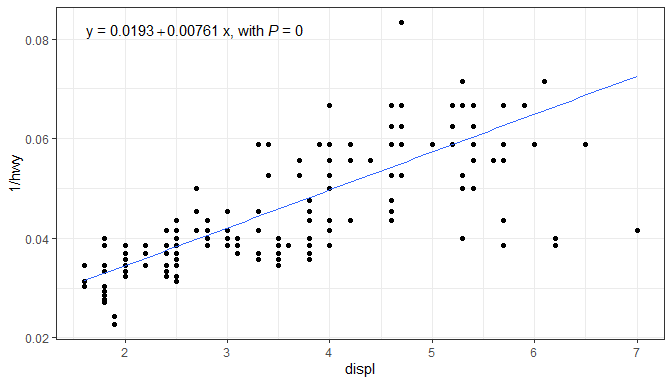We can define a stat_rq_eq() if we need to add similar equations to several plots. In this example we retain the ability of the user to override most of the default default arguments.

stat_rq_eqn <-
function(formula = y ~ x,
tau = 0.5,
method = "br",
mapping = aes(label = sprintf('y~"="~%.3g+%.3g~x*", with "*italic(P)~"="~%.3f',
after_stat(Intercept_estimate),
after_stat(x_estimate),
after_stat(x_p.value))),
parse = TRUE,
...) {
method.args <- list(formula = formula, tau = tau, method = method)
stat_fit_tidy(method = "rq",
method.args = method.args,
tidy.args = list(se.type = "nid"),
mapping = mapping,
parse = parse,
...)
}

And the code of the figure now as simple as. Figure not shown, as is identical to the one above.

ggplot(mpg, aes(displ, 1 / hwy)) +
geom_point() +
geom_quantile(quantiles = 0.5, formula = my_formula) +
stat_rq_eqn(tau = 0.5, formula = my_formula)### stat_fit_augment

Experimental! Use ggplot2::stat_smooth instead of stat_fit_augment if possible.

For a single panel and no grouping, there is little advantage in using this statistic compared to the examples in the documentation of package ‘broom’. With grouping and faceting stat_fit_augment may occasionally be more convenient than ggplot2::stat_smooth because of its additional flexibility.

# formula <- y ~ poly(x, 3, raw = TRUE)
# broom::augment does not handle poly correctly!
formula <- y ~ x + I(x^2) + I(x^3)
ggplot(my.data, aes(x, y)) +
geom_point() +
stat_fit_augment(method = "lm",
method.args = list(formula = formula))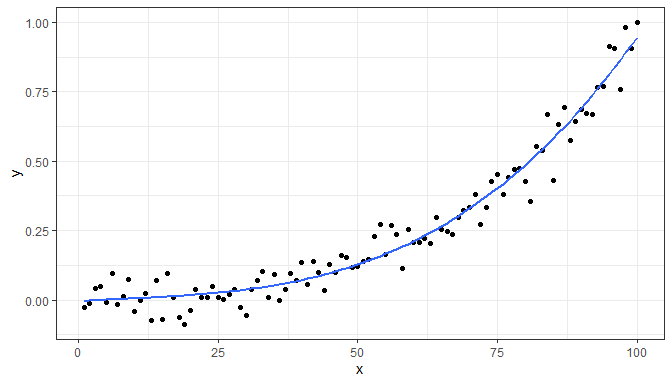formula <- y ~ x + I(x^2) + I(x^3)
ggplot(my.data, aes(x, y, colour = group)) +
geom_point() +
stat_fit_augment(method = "lm",
method.args = list(formula = formula))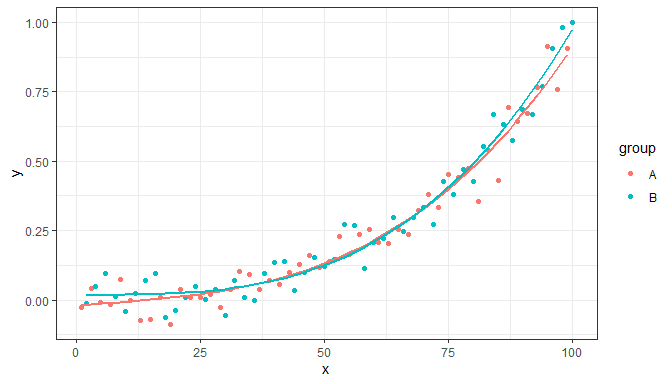We can override the variable returned as y to be any of the variables in the data frame returned by broom::augment while still preserving the original y values.

formula <- y ~ x + I(x^2) + I(x^3)
ggplot(my.data, aes(x, y)) +
stat_fit_augment(method = "lm",
method.args = list(formula = formula),
geom = "point",
y.out = ".resid")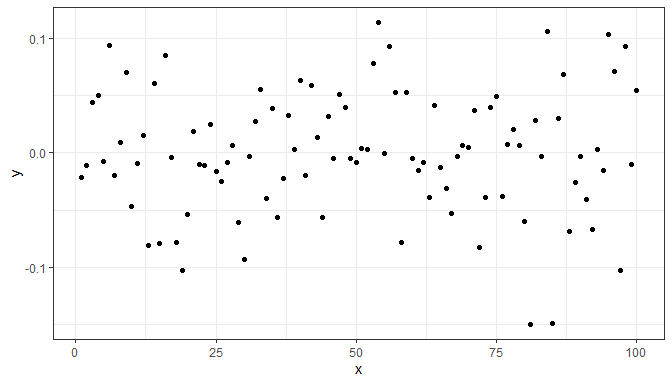formula <- y ~ x + I(x^2) + I(x^3)
ggplot(my.data, aes(x, y, colour = group)) +
stat_fit_augment(method = "lm",
method.args = list(formula = formula),
geom = "point",
y.out = ".std.resid")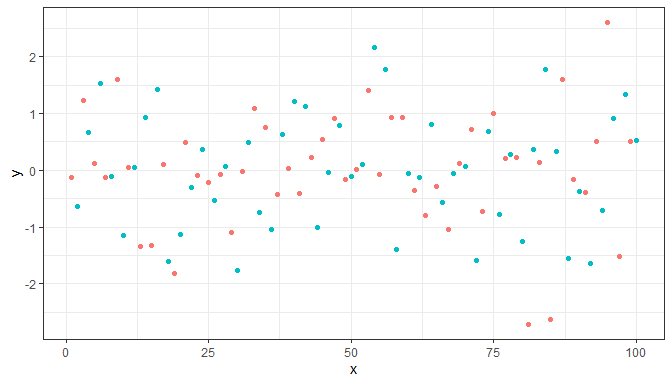We can use any model fitting method for which augment is implemented.

args <- list(formula = y ~ k * e ^ x,
start = list(k = 1, e = 2))
ggplot(mtcars, aes(wt, mpg)) +
geom_point() +
stat_fit_augment(method = "nls",
method.args = args)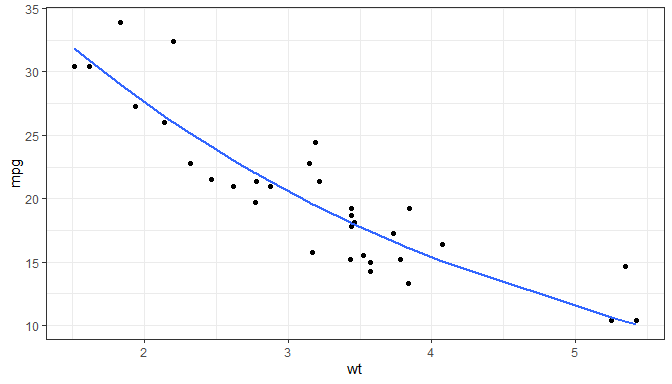args <- list(formula = y ~ k * e ^ x,
start = list(k = 1, e = 2))
ggplot(mtcars, aes(wt, mpg)) +
stat_fit_augment(method = "nls",
method.args = args,
geom = "point",
y.out = ".resid")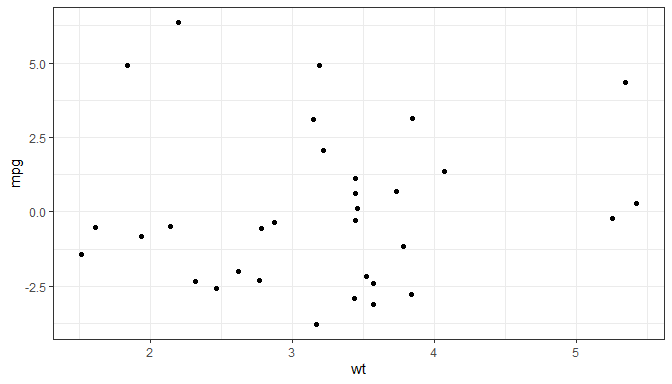Note: The tidiers for mixed models have moved to package ‘broom.mixed’!

args <- list(model = y ~ SSlogis(x, Asym, xmid, scal),
fixed = Asym + xmid + scal ~1,
random = Asym ~1 | group,
start = c(Asym = 200, xmid = 725, scal = 350))
ggplot(Orange, aes(age, circumference, colour = Tree)) +
geom_point() +
stat_fit_augment(method = "nlme",
method.args = args,
augment.args = list(data = quote(data)))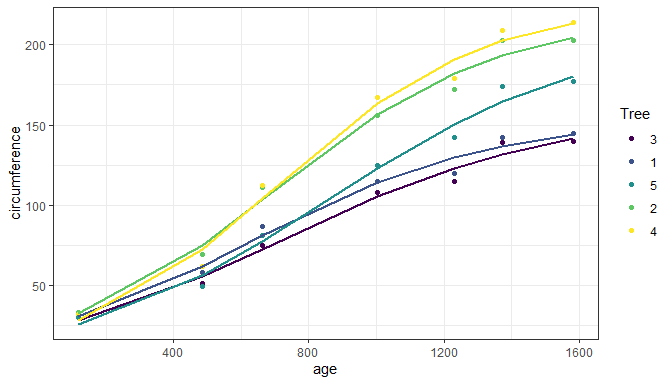## Scales

Volcano and quadrant plots are scatter plots with some peculiarities. Creating these plots from scratch using ‘ggplot2’ can be time consuming, but allows flexibility in design. Rather than providing a ‘canned’ function to produce volcano plots, package ‘ggpmisc’ provides several building blocks that facilitate the production of volcano and quadrant plots. These are wrappers on scales from packages ‘scales’ and ‘ggplot2’ with suitable defaults plus helper functions to create factors from numeric outcomes.

### scale_color_outcome, scale_fill_outcome

Manual scales for color and fill aesthetics with defaults suitable for the three way outcomes from statistical tests.

### scale_x_FDR, scale_y_FDR, scale_x_logFC, scale_y_logFC, scale_x_Pvalue, scale_y_Pvalue

Scales for x or y aesthetics mapped to P-values, FDR (false discovery rate) or log FC (logarithm of fold-change) as used in volcano and quadrant plots of transcriptomics and metabolomics data.

### symmetric_limits

A simple function to expand scale limits to be symmetric around zero. Can be passed as argument to parameter limits of continuous scales from packages ‘ggpmisc’, ‘ggplot2’ or ‘scales’ (and extensions).

### Volcano-plot examples

Volcano plots are frequently used for transcriptomics and metabolomics. They are used to show P-values or FDR (false discovery rate) as a function of effect size, which can be either an increase or a decrease. Effect sizes are expressed as fold-changes compared to a control or reference condition. Colors are frequently used to highlight significant responses. Counts of significant increases and decreases are frequently also added.

Outcomes encoded as -1, 0 or 1, as seen in the tibble below need to be converted into factors with suitable labels for levels. This can be easily achieved with function outcome2factor().

head(volcano_example.df) 
##         tag     gene outcome       logFC     PValue genotype
## 1 AT1G01040     ASU1       0 -0.15284466 0.35266997      Ler
## 2 AT1G01290     ASG4       0 -0.30057068 0.05471732      Ler
## 3 AT1G01560 ATSBT1.1       0 -0.57783350 0.06681310      Ler
## 4 AT1G01790   AtSAM1       0 -0.04729662 0.74054263      Ler
## 5 AT1G02130  AtTRM82       0 -0.14279891 0.29597519      Ler
## 6 AT1G02560    PRP39       0  0.23320752 0.07487043      Ler

Most frequently fold-change data is available log-transformed, using either 2 or 10 as base. In general it is more informative to use tick labels expressed as un-transformed fold-change.

By default scale_x_logFC() and scale_y_logFC() expect the logFC data log2-transformed, but this can be overridden. The default use of untransformed fold-change for tick labels can also be overridden. Scale scale_x_logFC() in addition by default expands the scale limits to make them symmetric around zero. If %unit is included in the name character string, suitable units are appended as shown in the example below.

Scales scale_y_Pvalue() and scale_x_Pvalue() have suitable defaults for name and labels, while scale_colour_outcome() provides suitable defaults for the colors mapped to the outcomes. To change the labels and title of the key or guide pass suitable arguments to parameters name and labels of these scales. These x and y scales by default squish off-limits (out-of-bounds) observations towards the limits.

volcano_example.df %>%
mutate(., outcome.fct = outcome2factor(outcome)) %>%
ggplot(., aes(logFC, PValue, colour = outcome.fct)) +
geom_point() +
scale_x_logFC(name = "Transcript abundance%unit") +
scale_y_Pvalue() +
scale_colour_outcome() +
stat_quadrant_counts(data = . %>% filter(outcome != 0))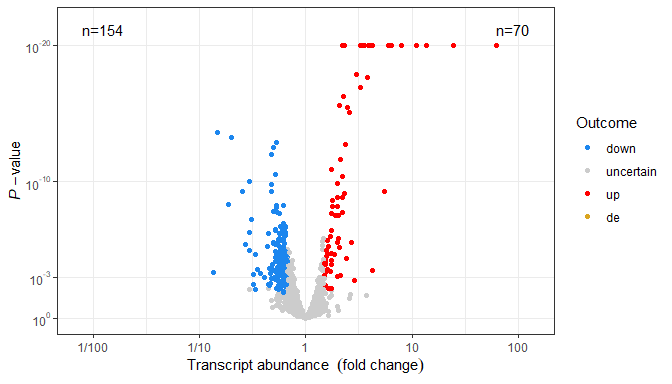By default outcome2factor() creates a factor with three levels as in the example above, but this default can be overridden as shown below.

volcano_example.df %>%
mutate(., outcome.fct = outcome2factor(outcome, n.levels = 2)) %>%
ggplot(., aes(logFC, PValue, colour = outcome.fct)) +
geom_point() +
scale_x_logFC(name = "Transcript abundance%unit") +
scale_y_Pvalue() +
scale_colour_outcome() +
stat_quadrant_counts(data = . %>% filter(outcome != 0))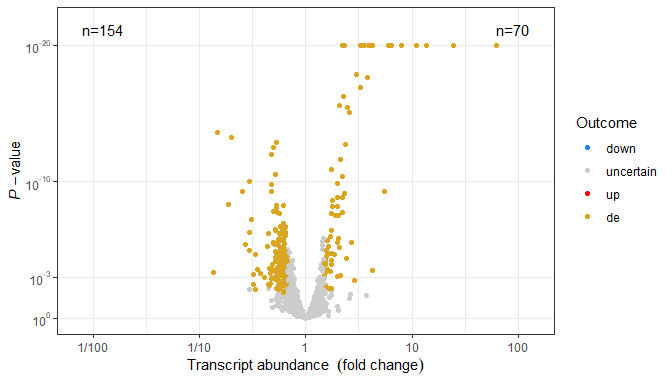Quadrant plots are scatter plots with comparable variables on both axes and usually include the four quadrants. When used for transcriptomics and metabolomics, they are used to compare responses expressed as fold-change to two different conditions or treatments. They are useful when many responses are measured as in transcriptomics (many different genes) or metabolomics (many different metabolites).

A single panel quadrant plot is as easy to produce as a volcano plot using scales scale_x_logFC() and scale_y_logFC(). The data includes two different outcomes and two different log fold-change variables. See the previous section on volcano plots for details. In this example we use as shape a filled circle and map one of the outcomes to color and the other to fill, using the two matched scales scale_colour_outcome() and scale_fill_outcome().

head(quadrant_example.df)
##         tag          gene outcome.x outcome.y    logFC.x     logFC.y genotype
## 1 AT5G12290         AtMC9         0         0 -0.3226849  0.32887081      Ler
## 2 AT5G47040        ATFRO2         0         0 -0.1067945 -0.18828653      Ler
## 3 AT5G57560 14-3-3EPSILON         0         0  0.2232457  0.74447768      Ler
## 4 AT5G24110       ATPTEN1         0         0 -0.7253487  0.06952586      Ler
## 5 AT2G41290         RHF2A         0         0  0.4435049 -0.32347741      Ler
## 6 AT4G36650          GAE5         0         0 -0.1410215  0.18697968      Ler

In this plot we do not include those genes whose change in transcript abundance is uncertain under both x and y conditions.

quadrant_example.df %>%
mutate(.,
outcome.x.fct = outcome2factor(outcome.x),
outcome.y.fct = outcome2factor(outcome.y),
outcome.xy.fct = xy_outcomes2factor(outcome.x, outcome.y)) %>%
filter(outcome.xy.fct != "none") %>%
ggplot(., aes(logFC.x, logFC.y, colour = outcome.x.fct, fill = outcome.y.fct)) +
geom_quadrant_lines(linetype = "dotted") +
stat_quadrant_counts(size = 3, colour = "white") +
geom_point(shape = "circle filled") +
scale_x_logFC(name = "Transcript abundance for x%unit") +
scale_y_logFC(name = "Transcript abundance for y%unit") +
scale_colour_outcome() +
scale_fill_outcome() +
theme_dark()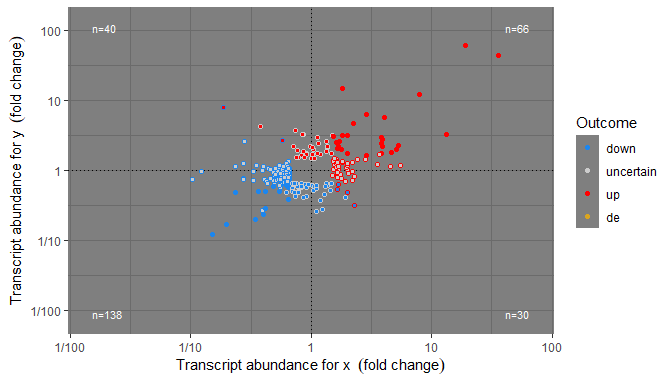To plot in separate panels those observations that are significant along both x and y axes, x axis, y axis, or none, with quadrants merged takes more effort. We first define two helper functions to add counts and quadrant lines to each of the four panels.

all_quadrant_counts <- function(...) {
list(
stat_quadrant_counts(data = . %>% filter(outcome.xy.fct == "xy"), ...),
stat_quadrant_counts(data = . %>% filter(outcome.xy.fct == "x"), pool.along = "y", ...),
stat_quadrant_counts(data = . %>% filter(outcome.xy.fct == "y"), pool.along = "x", ...),
stat_quadrant_counts(data = . %>% filter(outcome.xy.fct == "none"), quadrants = 0L, ...)
)
}
all_quadrant_lines <- function(...) {
list(
geom_hline(data =  data.frame(outcome.xy.fct = factor(c("xy", "x", "y", "none"),
levels = c("xy", "x", "y", "none")),
yintercept = c(0, NA, 0, NA)),
aes_(yintercept = ~yintercept),
na.rm = TRUE,
...),
geom_vline(data =  data.frame(outcome.xy.fct = factor(c("xy", "x", "y", "none"),
levels = c("xy", "x", "y", "none")),
xintercept = c(0, 0, NA, NA)),
aes_(xintercept = ~xintercept),
na.rm = TRUE,
...)
)
}

And use these functions to build the final plot, in this case including all genes.

quadrant_example.df %>%
mutate(.,
outcome.x.fct = outcome2factor(outcome.x),
outcome.y.fct = outcome2factor(outcome.y),
outcome.xy.fct = xy_outcomes2factor(outcome.x, outcome.y)) %>%
ggplot(., aes(logFC.x, logFC.y, colour = outcome.x.fct, fill = outcome.y.fct)) +
geom_point(shape = 21) +
all_quadrant_lines(linetype = "dotted") +
all_quadrant_counts(size = 3, colour = "white") +
scale_x_logFC(name = "Transcript abundance for x%unit") +
scale_y_logFC(name = "Transcript abundance for y%unit") +
scale_colour_outcome() +
scale_fill_outcome() +
facet_wrap(~outcome.xy.fct) +
theme_dark()